# StoichSubstanceSSTP Class Reference [Thermodynamic Properties]

Class StoichSubstanceSSTP represents a stoichiometric (fixed composition) incompressible substance. More...

#include <StoichSubstanceSSTP.h>

Inheritance diagram for StoichSubstanceSSTP: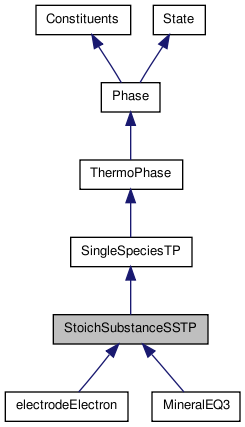[legend]
Collaboration diagram for StoichSubstanceSSTP: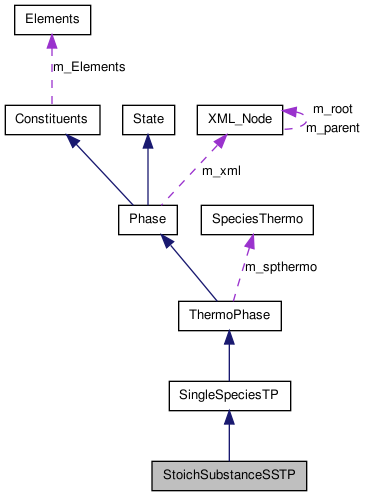[legend]

List of all members.

## Public Member Functions

StoichSubstanceSSTP ()
Default constructor for the StoichSubstanceSSTP class.
StoichSubstanceSSTP (std::string infile, std::string id="")
Construct and initialize a StoichSubstanceSSTP ThermoPhase object directly from an asci input file.
StoichSubstanceSSTP (XML_Node &phaseRef, std::string id="")
Construct and initialize a StoichSubstanceSSTP ThermoPhase object directly from an XML database.
StoichSubstanceSSTP (const StoichSubstanceSSTP &right)
Copy constructor.
StoichSubstanceSSTPoperator= (const StoichSubstanceSSTP &right)
Assignment operator.
virtual ~StoichSubstanceSSTP ()
Destructor for the routine (virtual).
ThermoPhaseduplMyselfAsThermoPhase () const
Duplication function.
doublereal _RT () const
Return the Gas Constant multiplied by the current temperature.
bool chargeNeutralityNecessary () const
Returns the chargeNeutralityNecessity boolean.
virtual std::string report (bool show_thermo=true) const
returns a summary of the state of the phase as a string
XML_Nodexml ()
Returns a reference to the XML_Node storred for the phase.
std::string id () const
Return the string id for the phase.
void setID (std::string id)
Set the string id for the phase.
std::string name () const
Return the name of the phase.
void setName (std::string nm)
Sets the string name for the phase.
void saveState (vector_fp &state) const
Save the current internal state of the phase.
void saveState (int lenstate, doublereal *state) const
Write to array 'state' the current internal state.
void restoreState (const vector_fp &state)
Restore a state saved on a previous call to saveState.
void restoreState (int lenstate, const doublereal *state)
Restore the state of the phase from a previously saved state vector.
void setMoleFractionsByName (compositionMap &xMap)
Set the species mole fractions by name.
void setMoleFractionsByName (const std::string &x)
Set the mole fractions of a group of species by name.
void setMassFractionsByName (compositionMap &yMap)
Set the species mass fractions by name.
void setMassFractionsByName (const std::string &x)
Set the species mass fractions by name.
void setState_TRX (doublereal t, doublereal dens, const doublereal *x)
Set the internally storred temperature (K), density, and mole fractions.
void setState_TRX (doublereal t, doublereal dens, compositionMap &x)
Set the internally storred temperature (K), density, and mole fractions.
void setState_TRY (doublereal t, doublereal dens, const doublereal *y)
Set the internally storred temperature (K), density, and mass fractions.
void setState_TRY (doublereal t, doublereal dens, compositionMap &y)
Set the internally storred temperature (K), density, and mass fractions.
void setState_TNX (doublereal t, doublereal n, const doublereal *x)
Set the internally storred temperature (K), molar density (kmol/m^3), and mole fractions.
void setState_TR (doublereal t, doublereal rho)
Set the internally storred temperature (K) and density (kg/m^3).
void setState_TX (doublereal t, doublereal *x)
Set the internally storred temperature (K) and mole fractions.
void setState_TY (doublereal t, doublereal *y)
Set the internally storred temperature (K) and mass fractions.
void setState_RX (doublereal rho, doublereal *x)
Set the density (kg/m^3) and mole fractions.
void setState_RY (doublereal rho, doublereal *y)
Set the density (kg/m^3) and mass fractions.
void getMolecularWeights (vector_fp &weights) const
Copy the vector of molecular weights into vector weights.
void getMolecularWeights (int iwt, doublereal *weights) const
Copy the vector of molecular weights into array weights.
void getMolecularWeights (doublereal *weights) const
Copy the vector of molecular weights into array weights.
const array_fp & molecularWeights () const
Return a const reference to the internal vector of molecular weights.
void getMoleFractionsByName (compositionMap &x) const
Get the mole fractions by name.
doublereal moleFraction (int k) const
Return the mole fraction of a single species.
doublereal moleFraction (std::string name) const
Return the mole fraction of a single species.
doublereal massFraction (int k) const
Return the mass fraction of a single species.
doublereal massFraction (std::string name) const
Return the mass fraction of a single species.
doublereal chargeDensity () const
Charge density [C/m^3].
int nDim () const
Returns the number of spatial dimensions (1, 2, or 3).
void setNDim (int ndim)
Set the number of spatial dimensions (1, 2, or 3).
virtual void freezeSpecies ()
Finished adding species, prepare to use them for calculation of mixture properties.
True if both elements and species have been frozen.
int nSpecies () const
Returns the number of species in the phase.
doublereal molecularWeight (int k) const
Molecular weight of species k.
doublereal molarMass (int k) const
Return the Molar mass of species k.
doublereal charge (int k) const
doublereal nAtoms (int k, int m) const
Number of atoms of element m in species k.
void getAtoms (int k, double *atomArray) const
Get a vector containing the atomic composition of species k.
void stateMFChangeCalc (bool forceChange=false)
Every time the mole fractions have changed, this routine will increment the stateMFNumber.
int stateMFNumber () const
Return the state number.
Utilities

virtual int eosType () const
Equation of state flag.
Mechanical Equation of State

virtual doublereal pressure () const
Report the Pressure. Units: Pa.
virtual void setPressure (doublereal p)
Set the pressure at constant temperature. Units: Pa.
virtual doublereal isothermalCompressibility () const
Returns the isothermal compressibility. Units: 1/Pa.
virtual doublereal thermalExpansionCoeff () const
Return the volumetric thermal expansion coefficient. Units: 1/K.
Activities, Standard States, and Activity Concentrations

This section is largely handled by parent classes, since there is only one species.

Therefore, the activity is equal to one.

virtual void getActivityConcentrations (doublereal *c) const
This method returns an array of generalized concentrations.
virtual doublereal standardConcentration (int k=0) const
Return the standard concentration for the kth species.
virtual doublereal logStandardConc (int k=0) const
Natural logarithm of the standard concentration of the kth species.
virtual void getStandardChemPotentials (doublereal *mu0) const
Get the array of chemical potentials at unit activity for the species at their standard states at the current T and P of the solution.
virtual void getUnitsStandardConc (doublereal *uA, int k=0, int sizeUA=6) const
Returns the units of the standard and generalized concentrations.
Properties of the Standard State of the Species in the Solution

virtual void getEnthalpy_RT (doublereal *hrt) const
Get the nondimensional Enthalpy functions for the species at their standard states at the current T and P of the solution.
virtual void getEntropy_R (doublereal *sr) const
Get the array of nondimensional Entropy functions for the standard state species at the current T and P of the solution.
virtual void getGibbs_RT (doublereal *grt) const
Get the nondimensional Gibbs functions for the species in their standard states at the current T and P of the solution.
virtual void getCp_R (doublereal *cpr) const
Get the nondimensional Heat Capacities at constant pressure for the species standard states at the current T and P of the solution.
virtual void getIntEnergy_RT (doublereal *urt) const
Returns the vector of nondimensional Internal Energies of the standard state species at the current T and P of the solution.
Thermodynamic Values for the Species Reference States

virtual void getIntEnergy_RT_ref (doublereal *urt) const
Returns the vector of nondimensional internal Energies of the reference state at the current temperature of the solution and the reference pressure for each species.
virtual void initThermo ()
virtual void initThermoXML (XML_Node &phaseNode, std::string id)
Import and initialize a ThermoPhase object using an XML tree.
virtual void setParameters (int n, doublereal *const c)
Set the equation of state parameters.
virtual void getParameters (int &n, doublereal *const c) const
Get the equation of state parameters in a vector.
virtual void setParametersFromXML (const XML_Node &eosdata)
Set equation of state parameter values from XML entries.
Molar Thermodynamic Properties of the Solution

These functions are resolved at this level, by reference to the partial molar functions and standard state functions for species 0.

Derived classes don't need to supply entries for these functions.

doublereal enthalpy_mole () const
Molar enthalpy. Units: J/kmol.
doublereal intEnergy_mole () const
Molar internal energy. Units: J/kmol.
doublereal entropy_mole () const
Molar entropy. Units: J/kmol/K.
doublereal gibbs_mole () const
Molar Gibbs function. Units: J/kmol.
doublereal cp_mole () const
Molar heat capacity at constant pressure. Units: J/kmol/K.
doublereal cv_mole () const
Molar heat capacity at constant volume. Units: J/kmol/K.
Activities, Standard State, and Activity Concentrations

The activity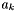of a species in solution is related to the chemical potential by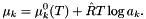The quantity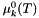is the chemical potential at unit activity, which depends only on temperature.

virtual void getActivities (doublereal *a) const
Get the array of non-dimensional activities at the current solution temperature, pressure, and solution concentration.
virtual void getActivityCoefficients (doublereal *ac) const
Get the array of non-dimensional activity coefficients at the current solution temperature, pressure, and solution concentration.
Partial Molar Properties of the Solution

These functions are resolved at this level, by reference to the partial molar functions and standard state functions for species 0.

Derived classes don't need to supply entries for these functions.

void getChemPotentials_RT (doublereal *murt) const
Get the array of non-dimensional species chemical potentials These are partial molar Gibbs free energies.
void getChemPotentials (doublereal *mu) const
Get the array of chemical potentials.
void getElectrochemPotentials (doublereal *mu) const
Get the species electrochemical potentials. Units: J/kmol.
void getPartialMolarEnthalpies (doublereal *hbar) const
Get the species partial molar enthalpies. Units: J/kmol.
virtual void getPartialMolarIntEnergies (doublereal *ubar) const
Get the species partial molar internal energies. Units: J/kmol.
void getPartialMolarEntropies (doublereal *sbar) const
Get the species partial molar entropy. Units: J/kmol K.
void getPartialMolarCp (doublereal *cpbar) const
Get the species partial molar Heat Capacities. Units: J/ kmol /K.
void getPartialMolarVolumes (doublereal *vbar) const
Get the species partial molar volumes. Units: m^3/kmol.
Properties of the Standard State of the Species in the Solution

These functions are the primary way real properties are supplied to derived thermodynamics classes of SingleSpeciesTP.

These functions must be supplied in derived classes. They are not resolved at the SingleSpeciesTP level.

void getPureGibbs (doublereal *gpure) const
Get the dimensional Gibbs functions for the standard state of the species at the current T and P.
void getStandardVolumes (doublereal *vbar) const
Get the molar volumes of each species in their standard states at the current T and P of the solution.
Thermodynamic Values for the Species Reference State

Almost all functions in this group are resolved by this class.

It is assumed that the m_spthermo species thermo pointer is populated and yields the reference state thermodynamics The internal energy function is not given by this class, since it would involve a specification of the equation of state.

virtual void getEnthalpy_RT_ref (doublereal *hrt) const
Returns the vector of nondimensional enthalpies of the reference state at the current temperature of the solution and the reference pressure for the species.
virtual void getGibbs_RT_ref (doublereal *grt) const
Returns the vector of nondimensional enthalpies of the reference state at the current temperature of the solution and the reference pressure for the species.
virtual void getGibbs_ref (doublereal *g) const
Returns the vector of the gibbs function of the reference state at the current temperature of the solution and the reference pressure for the species.
virtual void getEntropy_R_ref (doublereal *er) const
Returns the vector of nondimensional entropies of the reference state at the current temperature of the solution and the reference pressure for the species.
virtual void getCp_R_ref (doublereal *cprt) const
Get the nondimensional Gibbs functions for the standard state of the species at the current T and reference pressure for the species.
Setting the State

These methods set all or part of the thermodynamic state.

void setState_TPX (doublereal t, doublereal p, const doublereal *x)
Set the temperature (K), pressure (Pa), and mole fractions.
void setState_TPX (doublereal t, doublereal p, compositionMap &x)
Set the temperature (K), pressure (Pa), and mole fractions.
void setState_TPX (doublereal t, doublereal p, const std::string &x)
Set the temperature (K), pressure (Pa), and mole fractions.
void setState_TPY (doublereal t, doublereal p, const doublereal *y)
Set the internally storred temperature (K), pressure (Pa), and mass fractions of the phase.
void setState_TPY (doublereal t, doublereal p, compositionMap &y)
Set the internally storred temperature (K), pressure (Pa), and mass fractions of the phase.
void setState_TPY (doublereal t, doublereal p, const std::string &y)
Set the internally storred temperature (K), pressure (Pa), and mass fractions of the phase.
void setState_PX (doublereal p, doublereal *x)
Set the pressure (Pa) and mole fractions.
void setState_PY (doublereal p, doublereal *y)
Set the internally storred pressure (Pa) and mass fractions.
virtual void setState_HP (doublereal h, doublereal p, doublereal tol=1.e-8)
Set the internally storred specific enthalpy (J/kg) and pressure (Pa) of the phase.
virtual void setState_UV (doublereal u, doublereal v, doublereal tol=1.e-8)
Set the specific internal energy (J/kg) and specific volume (m^3/kg).
virtual void setState_SP (doublereal s, doublereal p, doublereal tol=1.e-8)
Set the specific entropy (J/kg/K) and pressure (Pa).
virtual void setState_SV (doublereal s, doublereal v, doublereal tol=1.e-8)
Set the specific entropy (J/kg/K) and specific volume (m^3/kg).
Saturation properties.

These methods are only implemented by subclasses that implement full liquid-vapor equations of state.

virtual doublereal satTemperature (doublereal p) const
Return the saturation temperature given the pressure.
virtual doublereal satPressure (doublereal t) const
Return the saturation pressure given the temperature.
virtual doublereal vaporFraction () const
Return the fraction of vapor at the current conditions.
virtual void setState_Tsat (doublereal t, doublereal x)
Set the state to a saturated system at a particular temperature.
virtual void setState_Psat (doublereal p, doublereal x)
Set the state to a saturated system at a particular pressure.
Information Methods

doublereal refPressure () const
Returns the reference pressure in Pa.
doublereal minTemp (int k=-1) const
Minimum temperature for which the thermodynamic data for the species or phase are valid.
doublereal Hf298SS (const int k) const
Report the 298 K Heat of Formation of the standard state of one species (J kmol-1).
virtual void modifyOneHf298SS (const int k, const doublereal Hf298New)
Modify the value of the 298 K Heat of Formation of one species in the phase (J kmol-1).
doublereal maxTemp (int k=-1) const
Maximum temperature for which the thermodynamic data for the species are valid.
Molar Thermodynamic Properties of the Solution

virtual void getdlnActCoeffdlnC (doublereal *dlnActCoeffdlnC) const
Get the array of log concentration-like derivatives of the log activity coefficients.
Mechanical Properties

virtual void updateDensity ()
Electric Potential

The phase may be at some non-zero electrical potential.

These methods set or get the value of the electric potential.

void setElectricPotential (doublereal v)
Set the electric potential of this phase (V).
doublereal electricPotential () const
Returns the electric potential of this phase (V).
Activities, Standard States, and Activity Concentrations

The activityof a species in solution is related to the chemical potential by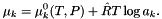The quantity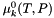is the standard chemical potential at unit activity, which depends on temperature and pressure, but not on composition.

The activity is dimensionless.

virtual int activityConvention () const
This method returns the convention used in specification of the activities, of which there are currently two, molar- and molality-based conventions.
virtual int standardStateConvention () const
This method returns the convention used in specification of the standard state, of which there are currently two, temperature based, and variable pressure based.
virtual void getUnitsStandardConc (double *uA, int k=0, int sizeUA=6) const
Returns the units of the standard and generalized concentrations.
virtual void getLNActivityCoefficients (doublereal *const lnac) const
Thermodynamic Values for the Species Reference States

virtual void getStandardVolumes_ref (doublereal *vol) const
Get the molar volumes of the species reference states at the current T and P_ref of the solution.
virtual void setReferenceComposition (const doublereal *const x)
Sets the reference composition.
virtual void getReferenceComposition (doublereal *const x) const
Gets the reference composition.
Specific Properties

doublereal enthalpy_mass () const
Specific enthalpy.
doublereal intEnergy_mass () const
Specific internal energy.
doublereal entropy_mass () const
Specific entropy.
doublereal gibbs_mass () const
Specific Gibbs function.
doublereal cp_mass () const
Specific heat at constant pressure.
doublereal cv_mass () const
Specific heat at constant volume.
Setting the State

These methods set all or part of the thermodynamic state.

void setState_TP (doublereal t, doublereal p)
Set the temperature (K) and pressure (Pa).
Chemical Equilibrium

Chemical equilibrium.

virtual void setToEquilState (const doublereal *lambda_RT)
This method is used by the ChemEquil equilibrium solver.
void setElementPotentials (const vector_fp &lambda)
Stores the element potentials in the ThermoPhase object.
bool getElementPotentials (doublereal *lambda) const
Returns the element potentials storred in the ThermoPhase object.
Critical State Properties.

These methods are only implemented by some subclasses, and may be moved out of ThermoPhase at a later date.

virtual doublereal critTemperature () const
Critical temperature (K).
virtual doublereal critPressure () const
Critical pressure (Pa).
virtual doublereal critDensity () const
Critical density (kg/m3).
Initialization Methods - For Internal Use (ThermoPhase)

void saveSpeciesData (const int k, const XML_Node *const data)
Store a reference pointer to the XML tree containing the species data for this phase.
const std::vector< const
XML_Node * > &
speciesData () const
Return a pointer to the vector of XML nodes containing the species data for this phase.
void setSpeciesThermo (SpeciesThermo *spthermo)
Install a species thermodynamic property manager.
SpeciesThermospeciesThermo ()
Return a changeable reference to the calculation manager for species reference-state thermodynamic properties.
virtual void initThermoFile (std::string inputFile, std::string id)
int index () const
void setIndex (int m)
virtual void setStateFromXML (const XML_Node &state)
Set the initial state of the phase to the conditions specified in the state XML element.
Element Information

std::string elementName (int m) const
Name of the element with index m.
int elementIndex (std::string name) const
Index of element named 'name'.
doublereal atomicWeight (int m) const
Atomic weight of element m.
doublereal entropyElement298 (int m) const
Entropy of the element in its standard state at 298 K and 1 bar.
int atomicNumber (int m) const
Atomic number of element m.
const std::vector< std::string > & elementNames () const
Return a read-only reference to the vector of element names.
const vector_fp & atomicWeights () const
Return a read-only reference to the vector of atomic weights.
int nElements () const
Number of elements.

These methods are used to add new elements or species.

These are not usually called by user programs.

Since species are checked to insure that they are only composed of declared elements, it is necessary to first add all elements before adding any species.

void addElement (const std::string &symbol, doublereal weight)
Add an element from an XML specification.
void addUniqueElement (const std::string &symbol, doublereal weight, int atomicNumber=0, doublereal entropy298=ENTROPY298_UNKNOWN)
Add an element, checking for uniqueness.
Adde an element, checking for uniqueness.
Add all elements referenced in an XML_Node tree.
void freezeElements ()
bool elementsFrozen ()
True if freezeElements has been called.

These methods are used to add new species.

They are not usually called by user programs.

void addSpecies (const std::string &name, const doublereal *comp, doublereal charge=0.0, doublereal size=1.0)
void addUniqueSpecies (const std::string &name, const doublereal *comp, doublereal charge=0.0, doublereal size=1.0)
Add a species to the phase, checking for uniqueness of the name.
int speciesIndex (std::string name) const
Index of species named 'name'.
std::string speciesName (int k) const
Name of the species with index k.
const std::vector< std::string > & speciesNames () const
Return a const referernce to the vector of species names.
doublereal size (int k) const
This routine returns the size of species k.
bool speciesFrozen ()
True if freezeSpecies has been called.
void clear ()
Remove all elements and species.
Composition

void getMoleFractions (doublereal *const x) const
Get the species mole fraction vector.
virtual void setMoleFractions (const doublereal *const x)
Set the mole fractions to the specified values, and then normalize them so that they sum to 1.0.
virtual void setMoleFractions_NoNorm (const doublereal *const x)
Set the mole fractions to the specified values without normalizing.
void getMassFractions (doublereal *const y) const
Get the species mass fractions.
virtual void setMassFractions (const doublereal *const y)
Set the mass fractions to the specified values, and then normalize them so that they sum to 1.0.
virtual void setMassFractions_NoNorm (const doublereal *const y)
Set the mass fractions to the specified values without normalizing.
void getConcentrations (doublereal *const c) const
Get the species concentrations (kmol/m^3).
doublereal concentration (const int k) const
Concentration of species k.
virtual void setConcentrations (const doublereal *const conc)
Set the concentrations to the specified values within the phase.
const doublereal * massFractions () const
Returns a read-only pointer to the start of the massFraction array.
const doublereal * moleFractdivMMW () const
Returns a read-only pointer to the start of the moleFraction/MW array.
Mean Properties

doublereal mean_X (const doublereal *const Q) const
Evaluate the mole-fraction-weighted mean of Q: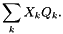Array Q should contain pure-species molar property values.

doublereal mean_Y (const doublereal *const Q) const
Evaluate the mass-fraction-weighted mean of Q: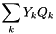.

doublereal meanMolecularWeight () const
The mean molecular weight.
doublereal sum_xlogx () const
Evaluate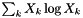.
doublereal sum_xlogQ (doublereal *const Q) const
Evaluate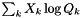.
Thermodynamic Properties

Class State only stores enough thermodynamic data to specify the state.

In addition to composition information, it stores the temperature and mass density.

doublereal temperature () const
Temperature (K).
virtual doublereal density () const
Density (kg/m^3).
doublereal molarDensity () const
Molar density (kmol/m^3).
virtual void setDensity (const doublereal density)
Set the internally storred density (kg/m^3) of the phase.
virtual void setMolarDensity (const doublereal molarDensity)
Set the internally storred molar density (kmol/m^3) of the phase.
virtual void setTemperature (const doublereal temp)
Set the temperature (K).

## Protected Member Functions

void _updateThermo () const
void init (const array_fp &mw)
void setMolecularWeight (const int k, const double mw)
Set the molecular weight of a single species to a given value.

## Protected Attributes

doublereal m_tmin
Lower value of the temperature for which reference thermo is valid.
doublereal m_tmax
Upper value of the temperature for which reference thermo is valid.
doublereal m_press
The current pressure of the solution (Pa).
doublereal m_p0
doublereal m_tlast
Last temperature used to evaluate the thermodynamic polynomial.
array_fp m_h0_RT
Dimensionless enthalpy at the (mtlast, m_p0).
array_fp m_cp0_R
Dimensionless heat capacity at the (mtlast, m_p0).
array_fp m_s0_R
Dimensionless entropy at the (mtlast, m_p0).
SpeciesThermom_spthermo
Pointer to the calculation manager for species reference-state thermodynamic properties.
std::vector< const XML_Node * > m_speciesData
Vector of pointers to the species databases.
int m_index
Index number of the phase.
doublereal m_phi
Storred value of the electric potential for this phase.
vector_fp m_lambdaRRT
Vector of element potentials.
bool m_hasElementPotentials
Boolean indicating whether there is a valid set of saved element potentials for this phase.
bool m_chargeNeutralityNecessary
Boolean indicating whether a charge neutrality condition is a necessity.
int m_ssConvention
Contains the standard state convention.
std::vector< doublereal > xMol_Ref
Reference Mole Fraction Composition.
int m_kk
m_kk = Number of species in the phase.
int m_ndim
m_ndim is the dimensionality of the phase.
vector_fp m_weight
Vector of molecular weights of the species.
bool m_speciesFrozen
Boolean indicating whether the number of species has been frozen.
Elementsm_Elements
std::vector< std::string > m_speciesNames
Vector of the species names.
vector_fp m_speciesComp
Atomic composition of the species.
vector_fp m_speciesCharge
m_speciesCharge: Vector of species charges length = m_kk
vector_fp m_speciesSize
m_speciesSize(): Vector of species sizes.

## Detailed Description

Class StoichSubstanceSSTP represents a stoichiometric (fixed composition) incompressible substance.

This class internally changes the independent degree of freedom from density to pressure. This is necessary because the phase is incompressible. It uses a constant volume approximation.

Specification of Species Standard State Properties

This class inherits from SingleSpeciesTP. It is assumed that the reference state thermodynamics may be obtained by a pointer to a populated species thermodynamic property manager class (see ThermoPhase::m_spthermo). How to relate pressure changes to the reference state thermodynamics is resolved at this level.

For an incompressible, stoichiometric substance, the molar internal energy is independent of pressure. Since the thermodynamic properties are specified by giving the standard-state enthalpy, the term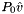is subtracted from the specified molar enthalpy to compute the molar internal energy. The entropy is assumed to be independent of the pressure.

The enthalpy function is given by the following relation.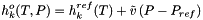For an incompressible, stoichiometric substance, the molar internal energy is independent of pressure. Since the thermodynamic properties are specified by giving the standard-state enthalpy, the term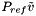is subtracted from the specified reference molar enthalpy to compute the molar internal energy.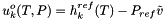The standard state heat capacity and entropy are independent of pressure. The standard state gibbs free energy is obtained from the enthalpy and entropy functions.

Specification of Solution Thermodynamic Properties

All solution properties are obtained from the standard state species functions, since there is only one species in the phase.

Application within Kinetics Managers

The standard concentration is equal to 1.0. This means that the kinetics operator works on an (activities basis). Since this is a stoichiometric substance, this means that the concentration of this phase drops out of kinetics expressions.

An example of a reaction using this is a sticking coefficient reaction of a substance in an ideal gas phase on a surface with a bulk phase species in this phase. In this case, the rate of progress for this reaction,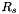, may be expressed via the following equation: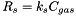where the units forare kmol m-2 s-1.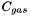has units of kmol m-3. Therefore, the kinetic rate constant,, has units of m s-1. Nowhere does the concentration of the bulk phase appear in the rate constant expression, since it's a stoichiometric phase and the activity is always equal to 1.0.

Instanteation of the Class

The constructor for this phase is NOT located in the default ThermoFactory for Cantera. However, a new StoichSubstanceSSTP may be created by the following code snippets:

    sprintf(file_ID,"%s#NaCl(S)", iFile);
XML_Node *xm = get_XML_NameID("phase", file_ID, 0);
StoichSubstanceSSTP *solid = new StoichSubstanceSSTP(*xm);


or by the following call to importPhase():

    sprintf(file_ID,"%s#NaCl(S)", iFile);
XML_Node *xm = get_XML_NameID("phase", file_ID, 0);
StoichSubstanceSSTP solid;
importPhase(*xm, &solid);


XML Example

The phase model name for this is called StoichSubstance. It must be supplied as the model attribute of the thermo XML element entry. Within the phase XML block, the density of the phase must be specified. An example of an XML file this phase is given below.

     <!-- phase NaCl(S)    -->
<phase dim="3" id="NaCl(S)">
<elementArray datasrc="elements.xml">
Na Cl
</elementArray>
<speciesArray datasrc="#species_NaCl(S)"> NaCl(S) </speciesArray>
<thermo model="StoichSubstanceSSTP">
<density units="g/cm3">2.165</density>
</thermo>
<transport model="None"/>
<kinetics model="none"/>
</phase>

<!-- species definitions     -->
<speciesData id="species_NaCl(S)">
<!-- species NaCl(S)   -->
<species name="NaCl(S)">
<atomArray> Na:1 Cl:1 </atomArray>
<thermo>
<Shomate Pref="1 bar" Tmax="1075.0" Tmin="250.0">
<floatArray size="7">
50.72389, 6.672267, -2.517167,
10.15934, -0.200675, -427.2115,
130.3973
</floatArray>
</Shomate>
</thermo>
<density units="g/cm3">2.165</density>
</species>
</speciesData>  

The model attribute, "StoichSubstanceSSTP", on the thermo element identifies the phase as being a StoichSubstanceSSTP object.

Definition at line 166 of file StoichSubstanceSSTP.h.

## Constructor & Destructor Documentation

 StoichSubstanceSSTP ( )

Default constructor for the StoichSubstanceSSTP class.

Definition at line 37 of file StoichSubstanceSSTP.cpp.

Referenced by StoichSubstanceSSTP::duplMyselfAsThermoPhase().

 StoichSubstanceSSTP ( std::string infile, std::string id = "" )

Construct and initialize a StoichSubstanceSSTP ThermoPhase object directly from an asci input file.

Parameters:
 infile name of the input file id name of the phase id in the file. If this is blank, the first phase in the file is used.

Definition at line 49 of file StoichSubstanceSSTP.cpp.

 StoichSubstanceSSTP ( XML_Node & phaseRef, std::string id = "" )

Construct and initialize a StoichSubstanceSSTP ThermoPhase object directly from an XML database.

Parameters:
 phaseRef XML node pointing to a StoichSubstanceSSTP description id Id of the phase.

Definition at line 74 of file StoichSubstanceSSTP.cpp.

References XML_Node::child(), and Cantera::importPhase().

 StoichSubstanceSSTP ( const StoichSubstanceSSTP & right )

Copy constructor.

Parameters:
 right Object to be copied

Definition at line 97 of file StoichSubstanceSSTP.cpp.

References StoichSubstanceSSTP::operator=().

 ~StoichSubstanceSSTP ( )  [virtual]

Destructor for the routine (virtual).

Definition at line 119 of file StoichSubstanceSSTP.cpp.

## Member Function Documentation

 doublereal _RT ( ) const [inline, inherited]

Return the Gas Constant multiplied by the current temperature.

The units are Joules kmol-1

Definition at line 1526 of file ThermoPhase.h.

References Cantera::GasConstant, and State::temperature().

 void _updateThermo ( ) const [protected, inherited]

For internal use only.

This crucial internal routine calls the species thermo update program to calculate new species Cp0, H0, and S0 whenever the temperature has changed.

Definition at line 637 of file SingleSpeciesTP.cpp.

 int activityConvention ( ) const [virtual, inherited]

This method returns the convention used in specification of the activities, of which there are currently two, molar- and molality-based conventions.

Currently, there are two activity conventions:

• Molar-based activities Unit activity of species at either a hypothetical pure solution of the species or at a hypothetical pure ideal solution at infinite dilution cAC_CONVENTION_MOLAR 0
• default
• Molality-based acvtivities (unit activity of solutes at a hypothetical 1 molal solution referenced to infinite dilution at all pressures and temperatures). cAC_CONVENTION_MOLALITY 1

Reimplemented in MolalityVPSSTP.

Definition at line 150 of file ThermoPhase.cpp.

References Cantera::cAC_CONVENTION_MOLAR.

Referenced by vcs_MultiPhaseEquil::reportCSV().

 void addElement ( const XML_Node & e )  [inherited]

Add an element from an XML specification.

Parameters:
 e Reference to the XML_Node where the element is described.

Definition at line 138 of file Constituents.cpp.

 void addElement ( const std::string & symbol, doublereal weight )  [inherited]

Parameters:
 symbol Atomic symbol std::string. weight Atomic mass in amu.

Definition at line 132 of file Constituents.cpp.

 void addElementsFromXML ( const XML_Node & phase )  [inherited]

Add all elements referenced in an XML_Node tree.

Parameters:
 phase Reference to the top XML_Node of a phase

Definition at line 169 of file Constituents.cpp.

 void addUniqueElement ( const XML_Node & e )  [inherited]

Adde an element, checking for uniqueness.

The uniqueness is checked by comparing the string symbol. If not unique, nothing is done.

Parameters:
 e Reference to the XML_Node where the element is described.

Definition at line 164 of file Constituents.cpp.

 void addUniqueElement ( const std::string & symbol, doublereal weight, int atomicNumber = 0, doublereal entropy298 = ENTROPY298_UNKNOWN )  [inherited]

Add an element, checking for uniqueness.

The uniqueness is checked by comparing the string symbol. If not unique, nothing is done.

Parameters:
 symbol String symbol of the element weight Atomic weight of the element (kg kmol-1). atomicNumber Atomic number of the element (unitless) entropy298 Entropy of the element at 298 K and 1 bar in its most stable form. The default is the value ENTROPY298_UNKNOWN, which is interpreted as an unknown, and if used will cause Cantera to throw an error.

Definition at line 157 of file Constituents.cpp.

 void addUniqueSpecies ( const std::string & name, const doublereal * comp, doublereal charge = 0.0, doublereal size = 1.0 )  [inherited]

Add a species to the phase, checking for uniqueness of the name.

This routine checks for uniqueness of the string name. It only adds the species if it is unique.

Parameters:
 name String name of the species comp Double vector containing the elemental composition of the species. charge Charge of the species. Defaults to zero. size Size of the species (meters). Defaults to 1 meter.

Definition at line 357 of file Constituents.cpp.

 int atomicNumber ( int m ) const [inherited]

Atomic number of element m.

Parameters:
 m Element index

Definition at line 117 of file Constituents.cpp.

References Elements::atomicNumber(), and Constituents::m_Elements.

 doublereal atomicWeight ( int m ) const [inherited]

Atomic weight of element m.

Parameters:
 m Element index

Definition at line 95 of file Constituents.cpp.

References Elements::atomicWeight(), and Constituents::m_Elements.

 const vector_fp & atomicWeights ( ) const [inherited]

Return a read-only reference to the vector of atomic weights.

Definition at line 109 of file Constituents.cpp.

References Elements::atomicWeights(), and Constituents::m_Elements.

 doublereal charge ( int k ) const [inherited]

Electrical charge of one species k molecule, divided by the magnitude of the electron charge (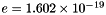Coulombs). Dimensionless.

Parameters:
 k species index

Definition at line 266 of file Constituents.cpp.

References Constituents::m_speciesCharge.

 doublereal chargeDensity ( ) const [inherited]

Charge density [C/m^3].

Definition at line 334 of file Phase.cpp.

References Constituents::charge(), Phase::moleFraction(), and Constituents::nSpecies().

 bool chargeNeutralityNecessary ( ) const [inline, inherited]

Returns the chargeNeutralityNecessity boolean.

Some phases must have zero net charge in order for their thermodynamics functions to be valid. If this is so, then the value returned from this function is true. If this is not the case, then this is false. Now, ideal gases have this parameter set to false, while solution with molality-based activity coefficients have this parameter set to true.

Definition at line 2066 of file ThermoPhase.h.

References ThermoPhase::m_chargeNeutralityNecessary.

 void clear ( )  [inherited]

Remove all elements and species.

 doublereal concentration ( const int k ) const [inherited]

Concentration of species k.

If k is outside the valid range, an exception will be thrown.

Parameters:
 k Index of species

Definition at line 134 of file State.cpp.

References State::m_dens, State::m_kk, State::m_rmolwts, and State::m_y.

 doublereal cp_mass ( ) const [inline, inherited]

Specific heat at constant pressure.

Units: J/kg/K.

Definition at line 1510 of file ThermoPhase.h.

References ThermoPhase::cp_mole(), and State::meanMolecularWeight().

 doublereal cp_mole ( ) const [virtual, inherited]

Molar heat capacity at constant pressure. Units: J/kmol/K.

This function is resolved here by calling the standard state thermo function.

Molar heat capacity at constant pressure of the mixture. Units: J/kmol/K.

Reimplemented from ThermoPhase.

Definition at line 183 of file SingleSpeciesTP.cpp.

References Cantera::GasConstant, and ThermoPhase::getCp_R().

Referenced by SingleSpeciesTP::cv_mole().

 virtual doublereal critDensity ( ) const [inline, virtual, inherited]

Critical density (kg/m3).

Reimplemented in HMWSoln, IdealMolalSoln, PureFluidPhase, and WaterSSTP.

Definition at line 1791 of file ThermoPhase.h.

References ThermoPhase::err().

 virtual doublereal critPressure ( ) const [inline, virtual, inherited]

Critical pressure (Pa).

Reimplemented in HMWSoln, IdealMolalSoln, PureFluidPhase, and WaterSSTP.

Definition at line 1786 of file ThermoPhase.h.

References ThermoPhase::err().

 virtual doublereal critTemperature ( ) const [inline, virtual, inherited]

Critical temperature (K).

Reimplemented in HMWSoln, IdealMolalSoln, PureFluidPhase, and WaterSSTP.

Definition at line 1781 of file ThermoPhase.h.

References ThermoPhase::err().

 doublereal cv_mass ( ) const [inline, inherited]

Specific heat at constant volume.

Units: J/kg/K.

Definition at line 1517 of file ThermoPhase.h.

References ThermoPhase::cv_mole(), and State::meanMolecularWeight().

 doublereal cv_mole ( ) const [virtual, inherited]

Molar heat capacity at constant volume. Units: J/kmol/K.

This function is resolved here by calling the standard state thermo function.

Reimplemented from ThermoPhase.

Definition at line 211 of file SingleSpeciesTP.cpp.

 virtual doublereal density ( ) const [inline, virtual, inherited]
 ThermoPhase * duplMyselfAsThermoPhase ( ) const [virtual]

Duplication function.

This virtual function is used to create a duplicate of the current phase. It's used to duplicate the phase when given a ThermoPhase pointer to the phase.

Returns:
It returns a ThermoPhase pointer.

Reimplemented from SingleSpeciesTP.

Reimplemented in MineralEQ3.

Definition at line 131 of file StoichSubstanceSSTP.cpp.

References StoichSubstanceSSTP::StoichSubstanceSSTP().

 doublereal electricPotential ( ) const [inline, inherited]

Returns the electric potential of this phase (V).

Units are Volts (which are Joules/coulomb)

Reimplemented in IdealMolalSoln.

Definition at line 1003 of file ThermoPhase.h.

References ThermoPhase::m_phi.

 int elementIndex ( std::string name ) const [inherited]

Index of element named 'name'.

The index is an integer assigned to each element in the order it was added, beginning with 0 for the first element.

Parameters:
 name name of the element

If 'name' is not the name of an element in the set, then the value -1 is returned.

Definition at line 197 of file Constituents.cpp.

References Elements::elementIndex(), and Constituents::m_Elements.

Referenced by MultiPhase::init(), WaterSSTP::initThermoXML(), and PDSS_HKFT::LookupGe().

 string elementName ( int m ) const [inherited]

Name of the element with index m.

This is a passthrough routine to the Element object.

Parameters:
 m Element index.
Exceptions:
 If m < 0 or m >= nElements(), the exception, ElementRangeError, is thrown.

Definition at line 209 of file Constituents.cpp.

References Elements::elementName(), and Constituents::m_Elements.

 const vector< string > & elementNames ( ) const [inherited]

Return a read-only reference to the vector of element names.

Definition at line 229 of file Constituents.cpp.

References Elements::elementNames(), and Constituents::m_Elements.

 bool elementsFrozen ( )  [inherited]

True if freezeElements has been called.

Definition at line 183 of file Constituents.cpp.

References Elements::elementsFrozen(), and Constituents::m_Elements.

 doublereal enthalpy_mass ( ) const [inline, inherited]

Specific enthalpy.

Units: J/kg.

Definition at line 1482 of file ThermoPhase.h.

References ThermoPhase::enthalpy_mole(), and State::meanMolecularWeight().

 doublereal enthalpy_mole ( ) const [virtual, inherited]

Molar enthalpy. Units: J/kmol.

------------ Molar Thermodynamic Properties --------------------

This function is resolved here by calling the standard state thermo function.

For this single species template, the molar properties of the mixture are identified with the partial molar properties of species number 0. The partial molar property routines are called to evaluate these functions. enthalpy_mole(): Molar enthalpy. Units: J/kmol.

Reimplemented from ThermoPhase.

Definition at line 133 of file SingleSpeciesTP.cpp.

References SingleSpeciesTP::getPartialMolarEnthalpies().

 doublereal entropy_mass ( ) const [inline, inherited]

Specific entropy.

Units: J/kg/K.

Definition at line 1496 of file ThermoPhase.h.

References ThermoPhase::entropy_mole(), and State::meanMolecularWeight().

 doublereal entropy_mole ( ) const [virtual, inherited]

Molar entropy. Units: J/kmol/K.

This function is resolved here by calling the standard state thermo function.

Molar entropy of the mixture. Units: J/kmol/K.

Reimplemented from ThermoPhase.

Definition at line 155 of file SingleSpeciesTP.cpp.

References SingleSpeciesTP::getPartialMolarEntropies().

 doublereal entropyElement298 ( int m ) const [inherited]

Entropy of the element in its standard state at 298 K and 1 bar.

Parameters:
 m Element index

Definition at line 100 of file Constituents.cpp.

References Elements::entropyElement298(), and Constituents::m_Elements.

Referenced by PDSS_HKFT::LookupGe().

 int eosType ( ) const [virtual]

Equation of state flag.

Returns the value cStoichSubstance, defined in mix_defs.h.

Reimplemented from SingleSpeciesTP.

Reimplemented in MineralEQ3.

Definition at line 145 of file StoichSubstanceSSTP.cpp.

 void freezeElements ( )  [inherited]

Definition at line 176 of file Constituents.cpp.

References Elements::freezeElements(), and Constituents::m_Elements.

 void freezeSpecies ( )  [virtual, inherited]

Finished adding species, prepare to use them for calculation of mixture properties.

Reimplemented from Constituents.

Definition at line 348 of file Phase.cpp.

 virtual void getActivities ( doublereal * a ) const [inline, virtual, inherited]

Get the array of non-dimensional activities at the current solution temperature, pressure, and solution concentration.

We redefine this function to just return 1.0 here.

Parameters:
 a Output vector of activities. Length: 1.

Reimplemented from ThermoPhase.

Definition at line 226 of file SingleSpeciesTP.h.

 virtual void getActivityCoefficients ( doublereal * ac ) const [inline, virtual, inherited]

Get the array of non-dimensional activity coefficients at the current solution temperature, pressure, and solution concentration.

Parameters:
 ac Output vector of activity coefficients. Length: 1.

Reimplemented from ThermoPhase.

Definition at line 237 of file SingleSpeciesTP.h.

References SingleSpeciesTP::err(), and Phase::m_kk.

 void getActivityConcentrations ( doublereal * c ) const [virtual]

This method returns an array of generalized concentrations.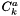are defined such that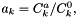where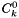is a standard concentration defined below andare activities used in the thermodynamic functions. These activity (or generalized) concentrations are used by kinetics manager classes to compute the forward and reverse rates of elementary reactions.

For a stoichiomeetric substance, there is only one species, and the generalized concentration is 1.0.

Parameters:
 c Output array of generalized concentrations. The units depend upon the implementation of the reaction rate expressions within the phase.

Reimplemented from ThermoPhase.

Reimplemented in MineralEQ3.

Definition at line 217 of file StoichSubstanceSSTP.cpp.

 void getAtoms ( int k, double * atomArray ) const [inherited]

Get a vector containing the atomic composition of species k.

Parameters:
 k species index atomArray vector containing the atomic number in the species. Length: m_mm

Definition at line 480 of file Constituents.cpp.

 void getChemPotentials ( doublereal * mu ) const [virtual, inherited]

Get the array of chemical potentials.

These are the phase, partial molar, and the standard state chemical potentials.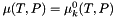.

Parameters:
 mu On return, Contains the chemical potential of the single species and the phase. Units are J / kmol . Length = 1

Reimplemented from ThermoPhase.

Definition at line 243 of file SingleSpeciesTP.cpp.

References ThermoPhase::getStandardChemPotentials().

Referenced by SingleSpeciesTP::getElectrochemPotentials(), and SingleSpeciesTP::gibbs_mole().

 void getChemPotentials_RT ( doublereal * murt ) const [virtual, inherited]

Get the array of non-dimensional species chemical potentials These are partial molar Gibbs free energies.

These are the phase, partial molar, and the standard state dimensionless chemical potentials.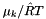.

Units: unitless

Parameters:
 murt On return, Contains the chemical potential / RT of the single species and the phase. Units are unitless. Length = 1

Reimplemented from ThermoPhase.

Definition at line 259 of file SingleSpeciesTP.cpp.

 void getConcentrations ( doublereal *const c ) const [inherited]

Get the species concentrations (kmol/m^3).

Parameters:
 c On return, c contains the concentrations for all species. Array c must have a length greater than or equal to the number of species.

Definition at line 219 of file State.cpp.

References State::m_dens, State::m_ym, and Cantera::scale().

 void getCp_R ( doublereal * cpr ) const [virtual]

Get the nondimensional Heat Capacities at constant pressure for the species standard states at the current T and P of the solution.

Parameters:
 cpr Output vector of nondimensional standard state heat capacities Length: m_kk.

Reimplemented from ThermoPhase.

Reimplemented in MineralEQ3.

Definition at line 329 of file StoichSubstanceSSTP.cpp.

References SingleSpeciesTP::_updateThermo(), and SingleSpeciesTP::m_cp0_R.

 void getCp_R_ref ( doublereal * cprt ) const [virtual, inherited]

Get the nondimensional Gibbs functions for the standard state of the species at the current T and reference pressure for the species.

Returns the vector of nondimensional constant pressure heat capacities of the reference state at the current temperature of the solution and reference pressure for each species.

This function is resolved in this class. It is assumed that the m_spthermo species thermo pointer is populated and yields the reference state.

Parameters:
 cprt Output vector of nondimensional reference state heat capacities at constant pressure for the species. Length: m_kk

Reimplemented from ThermoPhase.

Definition at line 444 of file SingleSpeciesTP.cpp.

References SingleSpeciesTP::_updateThermo(), and SingleSpeciesTP::m_cp0_R.

 virtual void getdlnActCoeffdlnC ( doublereal * dlnActCoeffdlnC ) const [inline, virtual, inherited]

Get the array of log concentration-like derivatives of the log activity coefficients.

This function is a virtual method. For ideal mixtures (unity activity coefficients), this can return zero. Implementations should take the derivative of the logarithm of the activity coefficient with respect to the logarithm of the concentration-like variable (i.e. mole fraction, molality, etc.) that represents the standard state. This quantity is to be used in conjunction with derivatives of that concentration-like variable when the derivative of the chemical potential is taken.

units = dimensionless

Parameters:
 dlnActCoeffdlnC Output vector of derivatives of the log Activity Coefficients. length = m_kk

Reimplemented in VPStandardStateTP.

Definition at line 904 of file ThermoPhase.h.

References ThermoPhase::err().

 void getElectrochemPotentials ( doublereal * mu ) const [inherited]

Get the species electrochemical potentials. Units: J/kmol.

This method adds a term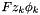to each chemical potential.

This is resolved here. A single species phase is not allowed to have anything other than a zero charge.

Parameters:
 mu On return, Contains the electrochemical potential of the single species and the phase. Units J/kmol . Length = 1

Reimplemented from ThermoPhase.

Definition at line 276 of file SingleSpeciesTP.cpp.

References SingleSpeciesTP::getChemPotentials().

 bool getElementPotentials ( doublereal * lambda ) const [inherited]

Returns the element potentials storred in the ThermoPhase object.

Returns the storred element potentials. The element potentials are retrieved from their storred dimensionless forms by multiplying by RT.

Parameters:
 lambda Output vector containing the element potentials. Length = nElements. Units are Joules/kmol.
Returns:
bool indicating whether thare are any valid storred element potentials. The calling routine should check this bool. In the case that there aren't any, lambda is not touched.

Definition at line 1015 of file ThermoPhase.cpp.

 void getEnthalpy_RT ( doublereal * hrt ) const [virtual]

Get the nondimensional Enthalpy functions for the species at their standard states at the current T and P of the solution.

Parameters:
 hrt Output vector of nondimensional standard state enthalpies. Length: m_kk.

Reimplemented from ThermoPhase.

Reimplemented in MineralEQ3.

Definition at line 299 of file StoichSubstanceSSTP.cpp.

Referenced by StoichSubstanceSSTP::getGibbs_RT().

 void getEnthalpy_RT_ref ( doublereal * hrt ) const [virtual, inherited]

Returns the vector of nondimensional enthalpies of the reference state at the current temperature of the solution and the reference pressure for the species.

Returns the vector of nondimensional enthalpies of the reference state at the current temperature of the solution and the reference pressure for the species.

This function is resolved in this class. It is assumed that the m_spthermo species thermo pointer is populated and yields the reference state.

Parameters:
 hrt Output vector containing the nondimensional reference state enthalpies Length: m_kk.

Reimplemented from ThermoPhase.

Definition at line 402 of file SingleSpeciesTP.cpp.

References SingleSpeciesTP::_updateThermo(), and SingleSpeciesTP::m_h0_RT.

 void getEntropy_R ( doublereal * sr ) const [virtual]

Get the array of nondimensional Entropy functions for the standard state species at the current T and P of the solution.

Parameters:
 sr Output vector of nondimensional standard state entropies. Length: m_kk.

Reimplemented from ThermoPhase.

Reimplemented in MineralEQ3.

Definition at line 311 of file StoichSubstanceSSTP.cpp.

References SingleSpeciesTP::getEntropy_R_ref().

 void getEntropy_R_ref ( doublereal * er ) const [virtual, inherited]

Returns the vector of nondimensional entropies of the reference state at the current temperature of the solution and the reference pressure for the species.

Returns the vector of nondimensional entropies of the reference state at the current temperature of the solution and the reference pressure for each species.

This function is resolved in this class. It is assumed that the m_spthermo species thermo pointer is populated and yields the reference state.

Parameters:
 er Output vector containing the nondimensional reference state entropies. Length: m_kk.

Reimplemented from ThermoPhase.

Definition at line 434 of file SingleSpeciesTP.cpp.

References SingleSpeciesTP::_updateThermo(), and SingleSpeciesTP::m_s0_R.

 void getGibbs_ref ( doublereal * g ) const [virtual, inherited]

Returns the vector of the gibbs function of the reference state at the current temperature of the solution and the reference pressure for the species.

Returns the vector of the gibbs function of the reference state at the current temperature of the solution and the reference pressure for the species. units = J/kmol

This function is resolved in this class. It is assumed that the m_spthermo species thermo pointer is populated and yields the reference state.

Parameters:
 g Output vector containing the reference state Gibbs Free energies. Length: m_kk. Units: J/kmol.

units = J/kmol

Reimplemented from ThermoPhase.

Definition at line 424 of file SingleSpeciesTP.cpp.

 void getGibbs_RT ( doublereal * grt ) const [virtual]

Get the nondimensional Gibbs functions for the species in their standard states at the current T and P of the solution.

Parameters:
 grt Output vector of nondimensional standard state gibbs free energies Length: m_kk.

Reimplemented from ThermoPhase.

Reimplemented in MineralEQ3.

Definition at line 320 of file StoichSubstanceSSTP.cpp.

References StoichSubstanceSSTP::getEnthalpy_RT(), and SingleSpeciesTP::m_s0_R.

Referenced by StoichSubstanceSSTP::getStandardChemPotentials().

 void getGibbs_RT_ref ( doublereal * grt ) const [virtual, inherited]

Returns the vector of nondimensional enthalpies of the reference state at the current temperature of the solution and the reference pressure for the species.

Returns the vector of nondimensional enthalpies of the reference state at the current temperature of the solution and the reference pressure for the species.

This function is resolved in this class. It is assumed that the m_spthermo species thermo pointer is populated and yields the reference state.

Parameters:
 grt Output vector containing the nondimensional reference state Gibbs Free energies. Length: m_kk.

Reimplemented from ThermoPhase.

Definition at line 413 of file SingleSpeciesTP.cpp.

Referenced by SingleSpeciesTP::getGibbs_ref(), and MetalSHEelectrons::getGibbs_RT().

 void getIntEnergy_RT ( doublereal * urt ) const [virtual]

Returns the vector of nondimensional Internal Energies of the standard state species at the current T and P of the solution.

For an incompressible, stoichiometric substance, the molar internal energy is independent of pressure. Since the thermodynamic properties are specified by giving the standard-state enthalpy, the term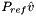is subtracted from the specified reference molar enthalpy to compute the standard state molar internal energy.

Parameters:
 urt output vector of nondimensional standard state internal energies of the species. Length: m_kk.

Reimplemented from ThermoPhase.

Reimplemented in MineralEQ3.

Definition at line 343 of file StoichSubstanceSSTP.cpp.

 void getIntEnergy_RT_ref ( doublereal * urt ) const [virtual]

Returns the vector of nondimensional internal Energies of the reference state at the current temperature of the solution and the reference pressure for each species.

Parameters:
 urt Output vector of nondimensional reference state internal energies of the species. Length: m_kk

Reimplemented from ThermoPhase.

Reimplemented in MineralEQ3.

Definition at line 366 of file StoichSubstanceSSTP.cpp.

 void getMassFractions ( doublereal *const y ) const [inherited]

Get the species mass fractions.

Parameters:
 y On return, y contains the mass fractions. Array y must have a length greater than or equal to the number of species. y Output vector of mass fractions. Length is m_kk.

Definition at line 235 of file State.cpp.

References State::m_y.

Referenced by ThermoPhase::report(), PureFluidPhase::report(), and Phase::saveState().

 void getMolecularWeights ( doublereal * weights ) const [inherited]

Copy the vector of molecular weights into array weights.

Parameters:
 weights Output array of molecular weights (kg/kmol)

Definition at line 289 of file Phase.cpp.

References Phase::molecularWeights().

 void getMolecularWeights ( int iwt, doublereal * weights ) const [inherited]

Copy the vector of molecular weights into array weights.

Parameters:
 iwt Unused. weights Output array of molecular weights (kg/kmol)
Deprecated:

Definition at line 281 of file Phase.cpp.

References Phase::molecularWeights().

 void getMolecularWeights ( vector_fp & weights ) const [inherited]

Copy the vector of molecular weights into vector weights.

Parameters:
 weights Output vector of molecular weights (kg/kmol)

Definition at line 271 of file Phase.cpp.

References Phase::molecularWeights().

 void getMoleFractions ( doublereal *const x ) const [inherited]

Get the species mole fraction vector.

Parameters:
 x On return, x contains the mole fractions. Must have a length greater than or equal to the number of species.

Definition at line 231 of file State.cpp.

References State::m_mmw, State::m_ym, and Cantera::scale().

 void getMoleFractionsByName ( compositionMap & x ) const [inherited]

Get the mole fractions by name.

Parameters:
 x Output composition map containing the species mole fractions.

Definition at line 306 of file Phase.cpp.

 void getParameters ( int & n, doublereal *const c ) const [virtual]

Get the equation of state parameters in a vector.

getParameters:

For internal use only.

Parameters:
 n number of parameters c array of n coefficients

For this phase:

• n = 1
• c = density of phase [ kg/m3 ]

Generic routine that is used to get the parameters used by this model. n = 1 C = density of phase [ kg/m3 ]

Reimplemented from SingleSpeciesTP.

Reimplemented in MineralEQ3.

Definition at line 462 of file StoichSubstanceSSTP.cpp.

References State::density().

 void getPartialMolarCp ( doublereal * cpbar ) const [virtual, inherited]

Get the species partial molar Heat Capacities. Units: J/ kmol /K.

This is the phase heat capacity.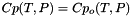.

This member function is resolved here. A single species phase obtains its thermo from the standard state function.

Parameters:
 cpbar On return, Contains the heat capacity of the single species and the phase. Units are J / kmol / K . Length = 1

Reimplemented from ThermoPhase.

Definition at line 337 of file SingleSpeciesTP.cpp.

References Cantera::GasConstant, and ThermoPhase::getCp_R().

 void getPartialMolarEnthalpies ( doublereal * hbar ) const [virtual, inherited]

Get the species partial molar enthalpies. Units: J/kmol.

These are the phase enthalpies.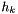.

This function is resolved here by calling the standard state thermo function.

Parameters:
 hbar Output vector of species partial molar enthalpies. Length: 1. units are J/kmol.

Reimplemented from ThermoPhase.

Definition at line 288 of file SingleSpeciesTP.cpp.

Referenced by SingleSpeciesTP::enthalpy_mole().

 void getPartialMolarEntropies ( doublereal * sbar ) const [virtual, inherited]

Get the species partial molar entropy. Units: J/kmol K.

This is the phase entropy.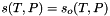.

This member function is resolved here. A single species phase obtains its thermo from the standard state function.

Parameters:
 sbar On return, Contains the entropy of the single species and the phase. Units are J / kmol / K . Length = 1

Reimplemented from ThermoPhase.

Definition at line 322 of file SingleSpeciesTP.cpp.

References Cantera::GasConstant, and ThermoPhase::getEntropy_R().

Referenced by SingleSpeciesTP::entropy_mole().

 void getPartialMolarIntEnergies ( doublereal * ubar ) const [virtual, inherited]

Get the species partial molar internal energies. Units: J/kmol.

These are the phase internal energies.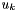.

This member function is resolved here. A single species phase obtains its thermo from the standard state function.

Parameters:
 ubar On return, Contains the internal energy of the single species and the phase. Units are J / kmol . Length = 1

Reimplemented from ThermoPhase.

Definition at line 305 of file SingleSpeciesTP.cpp.

Referenced by SingleSpeciesTP::intEnergy_mole().

 void getPartialMolarVolumes ( doublereal * vbar ) const [virtual, inherited]

Get the species partial molar volumes. Units: m^3/kmol.

This is the phase molar volume.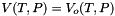.

This member function is resolved here. A single species phase obtains its thermo from the standard state function.

Parameters:
 vbar On return, Contains the molar volume of the single species and the phase. Units are m^3 / kmol. Length = 1

Reimplemented from ThermoPhase.

Definition at line 352 of file SingleSpeciesTP.cpp.

References State::density(), and Constituents::molecularWeight().

 void getPureGibbs ( doublereal * gpure ) const [virtual, inherited]

Get the dimensional Gibbs functions for the standard state of the species at the current T and P.

This function is resolved here by referencing getGibbs_RT().

Parameters:
 gpure returns a vector of size 1, containing the Gibbs function Units: J/kmol.

Reimplemented from ThermoPhase.

Definition at line 367 of file SingleSpeciesTP.cpp.

References Cantera::GasConstant, ThermoPhase::getGibbs_RT(), and State::temperature().

 void getReferenceComposition ( doublereal *const x ) const [virtual, inherited]

Gets the reference composition.

The reference mole fraction is a safe mole fraction.

Parameters:
 x Mole fraction vector containing the reference composition.

Definition at line 911 of file ThermoPhase.cpp.

References Phase::m_kk, and ThermoPhase::xMol_Ref.

 void getStandardChemPotentials ( doublereal * mu0 ) const [virtual]

Get the array of chemical potentials at unit activity for the species at their standard states at the current T and P of the solution.

For a stoichiometric substance, there is no activity term in the chemical potential expression, and therefore the standard chemical potential and the chemical potential are both equal to the molar Gibbs function.

These are the standard state chemical potentials. The values are evaluated at the current temperature and pressure of the solution

Parameters:
 mu0 Output vector of chemical potentials. Length: m_kk.

Reimplemented from ThermoPhase.

Reimplemented in MineralEQ3.

Definition at line 284 of file StoichSubstanceSSTP.cpp.

 void getStandardVolumes ( doublereal * vbar ) const [virtual, inherited]

Get the molar volumes of each species in their standard states at the current T and P of the solution.

units = m^3 / kmol

We resolve this function at this level, by assigning the molecular weight divided by the phase density

Parameters:
 vbar On output this contains the standard volume of the species and phase (m^3/kmol). Vector of length 1

Reimplemented from ThermoPhase.

Definition at line 384 of file SingleSpeciesTP.cpp.

References State::density(), and Constituents::molecularWeight().

 virtual void getStandardVolumes_ref ( doublereal * vol ) const [inline, virtual, inherited]

Get the molar volumes of the species reference states at the current T and P_ref of the solution.

units = m^3 / kmol

Parameters:
 vol Output vector containing the standard state volumes. Length: m_kk.

Reimplemented in IdealGasPhase, VPStandardStateTP, and WaterSSTP.

Definition at line 1449 of file ThermoPhase.h.

References ThermoPhase::err().

 void getUnitsStandardConc ( double * uA, int k = 0, int sizeUA = 6 ) const [virtual, inherited]

Returns the units of the standard and generalized concentrations.

Note they have the same units, as their ratio is defined to be equal to the activity of the kth species in the solution, which is unitless.

This routine is used in print out applications where the units are needed. Usually, MKS units are assumed throughout the program and in the XML input files.

The base ThermoPhase class assigns the default quantities of (kmol/m3) for all species. Inherited classes are responsible for overriding the default values if necessary.

Parameters:
 uA Output vector containing the units uA = kmol units - default = 1 uA = m units - default = -nDim(), the number of spatial dimensions in the Phase class. uA = kg units - default = 0; uA = Pa(pressure) units - default = 0; uA = Temperature units - default = 0; uA = time units - default = 0 k species index. Defaults to 0. sizeUA output int containing the size of the vector. Currently, this is equal to 6.

Reimplemented in DebyeHuckel, HMWSoln, IdealMolalSoln, IdealSolidSolnPhase, IdealSolnGasVPSS, and MolalityVPSSTP.

Definition at line 804 of file ThermoPhase.cpp.

References Phase::nDim().

 void getUnitsStandardConc ( doublereal * uA, int k = 0, int sizeUA = 6 ) const [virtual]

Returns the units of the standard and generalized concentrations.

Note they have the same units, as their ratio is defined to be equal to the activity of the kth species in the solution, which is unitless.

This routine is used in print out applications where the units are needed. Usually, MKS units are assumed throughout the program and in the XML input files.

The base ThermoPhase class assigns thedefault quantities of (kmol/m3) for all species. Inherited classes are responsible for overriding the default values if necessary.

Parameters:
 uA Output vector containing the units uA = kmol units - default = 1 uA = m units - default = -nDim(), the number of spatial dimensions in the Phase class. uA = kg units - default = 0; uA = Pa(pressure) units - default = 0; uA = Temperature units - default = 0; uA = time units - default = 0 k species index. Defaults to 0. sizeUA output int containing the size of the vector. Currently, this is equal to 6.

Reimplemented in MineralEQ3.

Definition at line 257 of file StoichSubstanceSSTP.cpp.

 doublereal gibbs_mass ( ) const [inline, inherited]

Specific Gibbs function.

Units: J/kg.

Definition at line 1503 of file ThermoPhase.h.

References ThermoPhase::gibbs_mole(), and State::meanMolecularWeight().

Referenced by ThermoPhase::report(), PureFluidPhase::report(), and MolalityVPSSTP::report().

 doublereal gibbs_mole ( ) const [virtual, inherited]

Molar Gibbs function. Units: J/kmol.

This function is resolved here by calling the standard state thermo function.

Molar Gibbs free energy of the mixture. Units: J/kmol/K.

Reimplemented from ThermoPhase.

Definition at line 166 of file SingleSpeciesTP.cpp.

References SingleSpeciesTP::getChemPotentials().

 doublereal Hf298SS ( const int k ) const [inline, inherited]

Report the 298 K Heat of Formation of the standard state of one species (J kmol-1).

The 298K Heat of Formation is defined as the enthalpy change to create the standard state of the species from its constituent elements in their standard states at 298 K and 1 bar.

Parameters:
 k species index
Returns:
Returns the current value of the Heat of Formation at 298K and 1 bar

Definition at line 816 of file ThermoPhase.h.

References ThermoPhase::err().

 std::string id ( ) const [inherited]

Return the string id for the phase.

Returns the id of the phase. The ID of the phase is set to the string name of the phase within the XML file Generally, it refers to the individual model name that denotes the species, the thermo, and the reaction rate info.

Definition at line 116 of file Phase.cpp.

References Phase::m_id.

Referenced by Kinetics::kineticsSpeciesIndex(), MultiPhase::phaseIndex(), and MultiPhase::phaseName().

 int index ( ) const [inline, inherited]

For internal use only.

Index number. This method can be used to identify the location of a phase object in a list, and is used by the interface library (clib) routines for this purpose.

Reimplemented from Phase.

Definition at line 1985 of file ThermoPhase.h.

References ThermoPhase::m_index.

 void init ( const array_fp & mw )  [protected, inherited]

For internal use only.

Initialize. Make a local copy of the vector of molecular weights, and resize the composition arrays to the appropriate size. The only information an instance of State has about the species is their molecular weights.

Parameters:
 mw Vector of molecular weights of the species.

Definition at line 244 of file State.cpp.

Referenced by Phase::freezeSpecies().

 void initThermo ( )  [virtual]

For internal use only.

Initialize. This method is provided to allow subclasses to perform any initialization required after all species have been added. For example, it might be used to resize internal work arrays that must have an entry for each species. The base class implementation does nothing, and subclasses that do not require initialization do not need to overload this method. When importing a CTML phase description, this method is called just prior to returning from function importPhase.

importCTML.cpp

Reimplemented from SingleSpeciesTP.

Reimplemented in MineralEQ3.

Definition at line 396 of file StoichSubstanceSSTP.cpp.

 void initThermoFile ( std::string inputFile, std::string id )  [virtual, inherited]

For internal use only.

Initialization of a ThermoPhase object using an ctml file.

This routine is a precursor to initThermoXML(XML_Node*) routine, which does most of the work. Here we read extra information about the XML description of a phase. Regular information about elements and species and their reference state thermodynamic information have already been read at this point. For example, we do not need to call this function for ideal gas equations of state.

Parameters:
 inputFile XML file containing the description of the phase id Optional parameter identifying the name of the phase. If none is given, the first XML phase element encountered will be used.

Definition at line 830 of file ThermoPhase.cpp.

 void initThermoXML ( XML_Node & phaseNode, std::string id )  [virtual]

Import and initialize a ThermoPhase object using an XML tree.

For internal use only.

Here we read extra information about the XML description of a phase. Regular information about elements and species and their reference state thermodynamic information have already been read at this point. For example, we do not need to call this function for ideal gas equations of state. This function is called from importPhase() after the elements and the species are initialized with default ideal solution level data.

The default implementation in ThermoPhase calls the virtual function initThermo() and then sets the "state" of the phase by looking for an XML element named "state", and then interpreting its contents by calling the virtual function setStateFromXML().

Parameters:
 phaseNode This object must be the phase node of a complete XML tree description of the phase, including all of the species data. In other words while "phase" must point to an XML phase object, it must have sibling nodes "speciesData" that describe the species in the phase. id ID of the phase. If nonnull, a check is done to see if phaseNode is pointing to the phase with the correct id.

Reimplemented from ThermoPhase.

Reimplemented in MineralEQ3, and electrodeElectron.

Definition at line 428 of file StoichSubstanceSSTP.cpp.

 doublereal intEnergy_mass ( ) const [inline, inherited]

Specific internal energy.

Units: J/kg.

Definition at line 1489 of file ThermoPhase.h.

References ThermoPhase::intEnergy_mole(), and State::meanMolecularWeight().

 doublereal intEnergy_mole ( ) const [virtual, inherited]

Molar internal energy. Units: J/kmol.

This function is resolved here by calling the standard state thermo function.

Reimplemented from ThermoPhase.

Definition at line 144 of file SingleSpeciesTP.cpp.

 doublereal isothermalCompressibility ( ) const [virtual]

Returns the isothermal compressibility. Units: 1/Pa.

The isothermal compressibility is defined as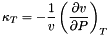Reimplemented from ThermoPhase.

Reimplemented in MineralEQ3.

Definition at line 188 of file StoichSubstanceSSTP.cpp.

 doublereal logStandardConc ( int k = 0 ) const [virtual]

Natural logarithm of the standard concentration of the kth species.

Parameters:
 k index of the species (defaults to zero)

Reimplemented from ThermoPhase.

Reimplemented in MineralEQ3.

Definition at line 234 of file StoichSubstanceSSTP.cpp.

 doublereal massFraction ( std::string name ) const [inherited]

Return the mass fraction of a single species.

Parameters:
 name String name of the species
Returns:
Mass Fraction of the species

Definition at line 328 of file Phase.cpp.

References State::massFractions(), and Constituents::speciesIndex().

 doublereal massFraction ( int k ) const [inherited]

Return the mass fraction of a single species.

Parameters:
 k String name of the species
Returns:
Mass Fraction of the species

Reimplemented from State.

Definition at line 324 of file Phase.cpp.

 const doublereal* massFractions ( ) const [inline, inherited]

Returns a read-only pointer to the start of the massFraction array.

Returns:
returns a pointer to a vector of doubles of length m_kk.

Definition at line 242 of file State.h.

References State::m_y.

Referenced by Phase::massFraction().

 doublereal maxTemp ( int k = -1 ) const [inline, inherited]

Maximum temperature for which the thermodynamic data for the species are valid.

If no argument is supplied, the value returned will be the highest temperature at which the data for all species are valid. Otherwise, the value will be only for species k. This function is a wrapper that calls the species thermo maxTemp function.

Parameters:
 k index of the species. Default is -1, which will return the min of the max value over all species.

Definition at line 845 of file ThermoPhase.h.

References ThermoPhase::m_spthermo, and SpeciesThermo::maxTemp().

 doublereal mean_X ( const doublereal *const Q ) const [inherited]

Evaluate the mole-fraction-weighted mean of Q:Array Q should contain pure-species molar property values.

Parameters:
 Q input vector of length m_kk that is to be averaged.
Returns:
mole-freaction-weighted mean of Q

Definition at line 223 of file State.cpp.

References State::m_mmw, and State::m_ym.

 doublereal mean_Y ( const doublereal *const Q ) const [inherited]

Evaluate the mass-fraction-weighted mean of Q:.

Parameters:
 Q Array Q contains a vector of species property values in mass units.
Returns:
Return value containing the mass-fraction-weighted mean of Q.

Definition at line 227 of file State.cpp.

References Cantera::dot(), and State::m_y.

 doublereal meanMolecularWeight ( ) const [inline, inherited]

The mean molecular weight.

Units: (kg/kmol)

Definition at line 282 of file State.h.

References State::m_mmw.

 doublereal minTemp ( int k = -1 ) const [inline, inherited]

Minimum temperature for which the thermodynamic data for the species or phase are valid.

If no argument is supplied, the value returned will be the lowest temperature at which the data for all species are valid. Otherwise, the value will be only for species k. This function is a wrapper that calls the species thermo minTemp function.

Parameters:
 k index of the species. Default is -1, which will return the max of the min value over all species.

Definition at line 776 of file ThermoPhase.h.

References ThermoPhase::m_spthermo, and SpeciesThermo::minTemp().

 virtual void modifyOneHf298SS ( const int k, const doublereal Hf298New )  [inline, virtual, inherited]

Modify the value of the 298 K Heat of Formation of one species in the phase (J kmol-1).

The 298K heat of formation is defined as the enthalpy change to create the standard state of the species from its constituent elements in their standard states at 298 K and 1 bar.

Parameters:
 k Species k Hf298New Specify the new value of the Heat of Formation at 298K and 1 bar

Definition at line 828 of file ThermoPhase.h.

References ThermoPhase::err().

 doublereal molarDensity ( ) const [inherited]
 doublereal molarMass ( int k ) const [inline, inherited]

Return the Molar mass of species k.

Preferred name for molecular weight.

Parameters:
 k index for species
Returns:
Return the molar mass of species k kg/kmol.

Definition at line 240 of file Constituents.h.

References Constituents::molecularWeight().

 doublereal molecularWeight ( int k ) const [inherited]

Molecular weight of species k.

Parameters:
 k index of species k
Returns:
Returns the molecular weight of species k.

Definition at line 240 of file Constituents.cpp.

References Constituents::m_weight, and Constituents::nSpecies().

 const array_fp & molecularWeights ( ) const [inherited]

Return a const reference to the internal vector of molecular weights.

Reimplemented from Constituents.

Definition at line 298 of file Phase.cpp.

Referenced by Phase::getMolecularWeights().

 const doublereal * moleFractdivMMW ( ) const [inherited]

Returns a read-only pointer to the start of the moleFraction/MW array.

This array is the array of mole fractions, each divided by the mean molecular weight.

Definition at line 215 of file State.cpp.

References State::m_ym.

 doublereal moleFraction ( std::string name ) const [inherited]

Return the mole fraction of a single species.

Parameters:
 name String name of the species
Returns:
Mole fraction of the species

Definition at line 318 of file Phase.cpp.

References Phase::moleFraction(), and Constituents::speciesIndex().

 doublereal moleFraction ( int k ) const [inherited]
 std::string name ( ) const [inherited]

Return the name of the phase.

Returns the name of the phase. The name of the phase is set to the string name of the phase within the XML file Generally, it refers to the individual model name that denotes the species, the thermo, and the reaction rate info. It may also refer more specifically to a location within the domain.

Definition at line 124 of file Phase.cpp.

References Phase::m_name.

 doublereal nAtoms ( int k, int m ) const [inherited]

Number of atoms of element m in species k.

Parameters:
 k species index m element index

Definition at line 463 of file Constituents.cpp.

 int nDim ( ) const [inline, inherited]

Returns the number of spatial dimensions (1, 2, or 3).

Definition at line 475 of file Phase.h.

References Phase::m_ndim.

 int nElements ( ) const [inherited]

Number of elements.

Definition at line 88 of file Constituents.cpp.

References Constituents::m_Elements, and Elements::nElements().

 int nSpecies ( ) const [inline, inherited]
 StoichSubstanceSSTP & operator= ( const StoichSubstanceSSTP & right )

Assignment operator.

Parameters:
 right Object to be copied

Definition at line 108 of file StoichSubstanceSSTP.cpp.

Referenced by StoichSubstanceSSTP::StoichSubstanceSSTP().

 doublereal pressure ( ) const [virtual]

Report the Pressure. Units: Pa.

----- Mechanical Equation of State ------

For an incompressible substance, the density is independent of pressure. This method simply returns the storred pressure value.

Reimplemented from ThermoPhase.

Reimplemented in MineralEQ3.

Definition at line 163 of file StoichSubstanceSSTP.cpp.

References SingleSpeciesTP::m_press.

 bool ready ( ) const [virtual, inherited]

True if both elements and species have been frozen.

Reimplemented from Constituents.

Definition at line 363 of file Phase.cpp.

 doublereal refPressure ( ) const [inline, inherited]

Returns the reference pressure in Pa.

This function is a wrapper that calls the species thermo refPressure function.

Definition at line 759 of file ThermoPhase.h.

References ThermoPhase::m_spthermo, and SpeciesThermo::refPressure().

 std::string report ( bool show_thermo = true ) const [virtual, inherited]

returns a summary of the state of the phase as a string

Parameters:
 show_thermo If true, extra information is printed out about the thermodynamic state of the system.

Reimplemented in MolalityVPSSTP, and PureFluidPhase.

Definition at line 1029 of file ThermoPhase.cpp.

 void restoreState ( int lenstate, const doublereal * state )  [inherited]

Restore the state of the phase from a previously saved state vector.

Parameters:
 lenstate Length of the state vector state Vector of state conditions.

Definition at line 154 of file Phase.cpp.

 void restoreState ( const vector_fp & state )  [inherited]

Restore a state saved on a previous call to saveState.

Parameters:
 state State vector containing the previously saved state.

Definition at line 150 of file Phase.cpp.

 virtual doublereal satPressure ( doublereal t ) const [inline, virtual, inherited]

Return the saturation pressure given the temperature.

Parameters:
 t Temperature (Kelvin)

Reimplemented from ThermoPhase.

Definition at line 683 of file SingleSpeciesTP.h.

References SingleSpeciesTP::err().

 virtual doublereal satTemperature ( doublereal p ) const [inline, virtual, inherited]

Return the saturation temperature given the pressure.

Parameters:
 p Pressure (Pa)

Reimplemented from ThermoPhase.

Definition at line 679 of file SingleSpeciesTP.h.

References SingleSpeciesTP::err().

 void saveSpeciesData ( const int k, const XML_Node *const data )  [inherited]

Store a reference pointer to the XML tree containing the species data for this phase.

The following methods are used in the process of constructing the phase and setting its parameters from a specification in an input file. They are not normally used in application programs. To see how they are used, see files importCTML.cpp and ThermoFactory.cpp.

This is used to access data needed to construct transport manager later.

For internal use only.

Parameters:
 k Species index data Pointer to the XML_Node data containing information about the species in the phase.

Definition at line 941 of file ThermoPhase.cpp.

References ThermoPhase::m_speciesData.

 void saveState ( int lenstate, doublereal * state ) const [inherited]

Write to array 'state' the current internal state.

Parameters:
 lenstate length of the state array. Must be >= nSpecies() + 2 state output vector. Must be of length nSpecies() + 2 or greater.

Definition at line 144 of file Phase.cpp.

References State::density(), State::getMassFractions(), and State::temperature().

 void saveState ( vector_fp & state ) const [inherited]

Save the current internal state of the phase.

Write to vector 'state' the current internal state.

Parameters:
 state output vector. Will be resized to nSpecies() + 2 on return.

Definition at line 140 of file Phase.cpp.

References Constituents::nSpecies().

 void setConcentrations ( const doublereal *const conc )  [virtual, inherited]

Set the concentrations to the specified values within the phase.

We set the concentrations here and therefore we set the overall density of the phase. We hold the temperature constant during this operation. Therefore, we have possibly changed the pressure of the phase by calling this routine.

Parameters:
 conc The input vector to this routine is in dimensional units. For volumetric phases c[k] is the concentration of the kth species in kmol/m3. For surface phases, c[k] is the concentration in kmol/m2. The length of the vector is the number of species in the phase.

Reimplemented in IdealSolidSolnPhase.

Definition at line 196 of file State.cpp.

Referenced by SurfPhase::setCoverages(), and SurfPhase::setCoveragesNoNorm().

 virtual void setDensity ( const doublereal density )  [inline, virtual, inherited]

Set the internally storred density (kg/m^3) of the phase.

Note the density of a phase is an indepedent variable.

Parameters:
 density Input density (kg/m^3).

Reimplemented in DebyeHuckel, HMWSoln, IdealMolalSoln, IdealSolidSolnPhase, and WaterSSTP.

Definition at line 327 of file State.h.

References State::m_dens.

 void setElectricPotential ( doublereal v )  [inline, inherited]

Set the electric potential of this phase (V).

This is used by classes InterfaceKinetics and EdgeKinetics to compute the rates of charge-transfer reactions, and in computing the electrochemical potentials of the species.

Each phase may have its own electric potential.

Parameters:
 v Input value of the electric potential in Volts

Reimplemented in IdealMolalSoln.

Definition at line 995 of file ThermoPhase.h.

References ThermoPhase::m_phi.

 void setElementPotentials ( const vector_fp & lambda )  [inherited]

Stores the element potentials in the ThermoPhase object.

Called by function 'equilibrate' in ChemEquil.h to transfer the element potentials to this object after every successful equilibration routine. The element potentials are storred in their dimensionless forms, calculated by dividing by RT.

Parameters:
 lambda Input vector containing the element potentials. Length = nElements. Units are Joules/kmol.

Definition at line 993 of file ThermoPhase.cpp.

 void setID ( std::string id )  [inherited]

Set the string id for the phase.

Sets the id of the phase. The ID of the phase is originally set to the string name of the phase within the XML file. Generally, it refers to the individual model name that denotes the species, the thermo, and the reaction rate info.

Parameters:
 id String id of the phase

Definition at line 120 of file Phase.cpp.

References Phase::m_id.

 void setIndex ( int m )  [inline, inherited]

For internal use only.

Set the index number. The Cantera interface library uses this method to set the index number to the location of the pointer to this object in the pointer array it maintains. Using this method for any other purpose will lead to unpredictable results if used in conjunction with the interface library.

Parameters:
 m Input the index number.

Reimplemented from Phase.

Definition at line 1998 of file ThermoPhase.h.

References ThermoPhase::m_index.

 void setMassFractions ( const doublereal *const y )  [virtual, inherited]

Set the mass fractions to the specified values, and then normalize them so that they sum to 1.0.

Parameters:
 y Array of unnormalized mass fraction values (input). Must have a length greater than or equal to the number of species. y Input vector of mass fractions. There is no restriction on the sum of the mass fraction vector. Internally, the State object will normalize this vector before storring its contents. Length is m_kk.

Reimplemented in IdealSolidSolnPhase.

Definition at line 142 of file State.cpp.

 void setMassFractions_NoNorm ( const doublereal *const y )  [virtual, inherited]

Set the mass fractions to the specified values without normalizing.

This is useful when the normalization condition is being handled by some other means, for example by a constraint equation as part of a larger set of equations.

Parameters:
 y Input vector of mass fractions. Length is m_kk.

Reimplemented in IdealSolidSolnPhase.

Definition at line 167 of file State.cpp.

Referenced by Phase::restoreState().

 void setMassFractionsByName ( const std::string & x )  [inherited]

Set the species mass fractions by name.

Species not listed by name in x are set to zero.

Parameters:
 x String containing a composition map

Definition at line 204 of file Phase.cpp.

 void setMassFractionsByName ( compositionMap & yMap )  [inherited]

Set the species mass fractions by name.

Parameters:
 yMap map from species names to mass fraction values. Species not listed by name in yMap are set to zero.

Definition at line 193 of file Phase.cpp.

 void setMolarDensity ( const doublereal molarDensity )  [virtual, inherited]

Set the internally storred molar density (kmol/m^3) of the phase.

Parameters:
 molarDensity Input molar density (kmol/m^3).

Reimplemented in DebyeHuckel, HMWSoln, IdealMolalSoln, and IdealSolidSolnPhase.

Definition at line 239 of file State.cpp.

References State::m_dens, and State::meanMolecularWeight().

Referenced by Phase::setState_TNX().

 void setMolecularWeight ( const int k, const double mw )  [inline, protected, inherited]

Set the molecular weight of a single species to a given value.

Parameters:
 k id of the species mw Molecular Weight (kg kmol-1)

Definition at line 385 of file State.h.

References State::m_molwts, and State::m_rmolwts.

 void setMoleFractions ( const doublereal *const x )  [virtual, inherited]

Set the mole fractions to the specified values, and then normalize them so that they sum to 1.0.

Parameters:
 x Array of unnormalized mole fraction values (input). Must have a length greater than or equal to the number of species. x Input vector of mole fractions. There is no restriction on the sum of the mole fraction vector. Internally, the State object will normalize this vector before storring its contents. Length is m_kk.

Reimplemented in IdealSolidSolnPhase.

Definition at line 102 of file State.cpp.

 void setMoleFractions_NoNorm ( const doublereal *const x )  [virtual, inherited]

Set the mole fractions to the specified values without normalizing.

This is useful when the normalization condition is being handled by some other means, for example by a constraint equation as part of a larger set of equations.

Parameters:
 x Input vector of mole fractions. Length is m_kk.

Reimplemented in IdealSolidSolnPhase.

Definition at line 115 of file State.cpp.

 void setMoleFractionsByName ( const std::string & x )  [inherited]

Set the mole fractions of a group of species by name.

The string x is in the form of a composition map Species which are not listed by name in the composition map are set to zero.

Parameters:
 x string x in the form of a composition map

Definition at line 177 of file Phase.cpp.

 void setMoleFractionsByName ( compositionMap & xMap )  [inherited]

Set the species mole fractions by name.

Parameters:
 xMap map from species names to mole fraction values. Species not listed by name in xMap are set to zero.

Definition at line 166 of file Phase.cpp.

 void setName ( std::string nm )  [inherited]

Sets the string name for the phase.

Sets the name of the phase. The name of the phase is originally set to the string name of the phase within the XML file. Generally, it refers to the individual model name that denotes the species, the thermo, and the reaction rate info. It may also refer more specifically to a location within the domain.

Parameters:
 nm String name of the phase

Definition at line 128 of file Phase.cpp.

References Phase::m_name.

 void setNDim ( int ndim )  [inline, inherited]

Set the number of spatial dimensions (1, 2, or 3).

The number of spatial dimensions is used for vector involving directions.

Parameters:
 ndim Input number of dimensions.

Definition at line 484 of file Phase.h.

References Phase::m_ndim.

Referenced by EdgePhase::EdgePhase(), EdgePhase::operator=(), and SurfPhase::SurfPhase().

 void setParameters ( int n, doublereal *const c )  [virtual]

Set the equation of state parameters.

setParameters:

For internal use only.

The number and meaning of these depends on the subclass.

Parameters:
 n number of parameters c array of n coefficients c = density of phase [ kg/m3 ]

Generic routine that is used to set the parameters used by this model. C = density of phase [ kg/m3 ]

Reimplemented from SingleSpeciesTP.

Reimplemented in MineralEQ3, and electrodeElectron.

Definition at line 449 of file StoichSubstanceSSTP.cpp.

References State::setDensity().

 void setParametersFromXML ( const XML_Node & eosdata )  [virtual]

Set equation of state parameter values from XML entries.

This method is called by function importPhase() in file importCTML.cpp when processing a phase definition in an input file. It should be overloaded in subclasses to set any parameters that are specific to that particular phase model. Note, this method is called before the phase is initialzed with elements and/or species.

For this phase, the density of the phase is specified in this block.

Parameters:
 eosdata An XML_Node object corresponding to the "thermo" entry for this phase in the input file.

eosdata points to the thermo block, and looks like this:

         <phase id="stoichsolid" >
<thermo model="StoichSubstance">
<density units="g/cm3">3.52</density>
</thermo>
</phase>    

Reimplemented from SingleSpeciesTP.

Reimplemented in MineralEQ3, and electrodeElectron.

Definition at line 479 of file StoichSubstanceSSTP.cpp.

References ctml::getFloat(), and State::setDensity().

 void setPressure ( doublereal p )  [virtual]

Set the pressure at constant temperature. Units: Pa.

For an incompressible substance, the density is independent of pressure. Therefore, this method only stores the specified pressure value. It does not modify the density.

Parameters:
 p Pressure (units - Pa)

Reimplemented from ThermoPhase.

Reimplemented in MineralEQ3.

Definition at line 174 of file StoichSubstanceSSTP.cpp.

References SingleSpeciesTP::m_press.

 void setReferenceComposition ( const doublereal *const x )  [virtual, inherited]

Sets the reference composition.

Parameters:
 x Mole fraction vector to set the reference composition to. If this is zero, then the reference mole fraction is set to the current mole fraction vector.

Definition at line 891 of file ThermoPhase.cpp.

References DATA_PTR, State::getMoleFractions(), Phase::m_kk, and ThermoPhase::xMol_Ref.

Referenced by ThermoPhase::initThermoXML().

 void setSpeciesThermo ( SpeciesThermo * spthermo )  [inline, inherited]

Install a species thermodynamic property manager.

The species thermodynamic property manager computes properties of the pure species for use in constructing solution properties. It is meant for internal use, and some classes derived from ThermoPhase may not use any species thermodynamic property manager. This method is called by function importPhase() in importCTML.cpp.

Parameters:
 spthermo input pointer to the species thermodynamic property manager.

For internal use only.

Definition at line 1887 of file ThermoPhase.h.

References ThermoPhase::m_spthermo.

Referenced by VPSSMgrFactory::newVPSSMgr().

 void setState_HP ( doublereal h, doublereal p, doublereal tol = 1.e-8 )  [virtual, inherited]

Set the internally storred specific enthalpy (J/kg) and pressure (Pa) of the phase.

Parameters:
 h Specific enthalpy (J/kg) p Pressure (Pa) tol Optional parameter setting the tolerance of the calculation.

Reimplemented from ThermoPhase.

Definition at line 498 of file SingleSpeciesTP.cpp.

 virtual void setState_Psat ( doublereal p, doublereal x )  [inline, virtual, inherited]

Set the state to a saturated system at a particular pressure.

Parameters:
 p Pressure (Pa) x Fraction of vapor

Reimplemented from ThermoPhase.

Definition at line 695 of file SingleSpeciesTP.h.

References SingleSpeciesTP::err().

 void setState_PX ( doublereal p, doublereal * x )  [inherited]

Set the pressure (Pa) and mole fractions.

Note, the mole fractions are set to X = 1.0. Setting the pressure may involve the solution of a nonlinear equation.

Parameters:
 p Pressure (Pa) x Vector of mole fractions. Length is equal to m_kk.

Reimplemented from ThermoPhase.

Definition at line 484 of file SingleSpeciesTP.cpp.

References SingleSpeciesTP::err(), and ThermoPhase::setPressure().

 void setState_PY ( doublereal p, doublereal * y )  [inherited]

Set the internally storred pressure (Pa) and mass fractions.

Note, the mass fractions are set to Y = 1.0. Note, the temperature is held constant during this operation. Setting the pressure may involve the solution of a nonlinear equation.

Parameters:
 p Pressure (Pa) y Vector of mass fractions. Length is equal to m_kk.

Reimplemented from ThermoPhase.

Definition at line 491 of file SingleSpeciesTP.cpp.

References SingleSpeciesTP::err(), and ThermoPhase::setPressure().

 void setState_RX ( doublereal rho, doublereal * x )  [inherited]

Set the density (kg/m^3) and mole fractions.

Parameters:
 rho Density (kg/m^3) x vector of species mole fractions. Length is equal to m_kk

Definition at line 259 of file Phase.cpp.

References State::setDensity(), and State::setMoleFractions().

 void setState_RY ( doublereal rho, doublereal * y )  [inherited]

Set the density (kg/m^3) and mass fractions.

Parameters:
 rho Density (kg/m^3) y vector of species mass fractions. Length is equal to m_kk

Definition at line 264 of file Phase.cpp.

References State::setDensity(), and State::setMassFractions().

 void setState_SP ( doublereal s, doublereal p, doublereal tol = 1.e-8 )  [virtual, inherited]

Set the specific entropy (J/kg/K) and pressure (Pa).

This function fixes the internal state of the phase so that the specific entropy and the pressure have the value of the input parameters.

Parameters:
 s specific entropy (J/kg/K) p specific pressure (Pa). tol Optional parameter setting the tolerance of the calculation.

Reimplemented from ThermoPhase.

Definition at line 532 of file SingleSpeciesTP.cpp.

 void setState_SV ( doublereal s, doublereal v, doublereal tol = 1.e-8 )  [virtual, inherited]

Set the specific entropy (J/kg/K) and specific volume (m^3/kg).

This function fixes the internal state of the phase so that the specific entropy and specific volume have the value of the input parameters.

Parameters:
 s specific entropy (J/kg/K) v specific volume (m^3/kg). tol Optional parameter setting the tolerance of the calculation.

Reimplemented from ThermoPhase.

Definition at line 548 of file SingleSpeciesTP.cpp.

 void setState_TNX ( doublereal t, doublereal n, const doublereal * x )  [inherited]

Set the internally storred temperature (K), molar density (kmol/m^3), and mole fractions.

Note, the mole fractions are always set first, before the molar density

Parameters:
 t Temperature in kelvin n molar density (kmol/m^3) x vector of species mole fractions. Length is equal to m_kk

Definition at line 220 of file Phase.cpp.

 void setState_TP ( doublereal t, doublereal p )  [inherited]

Set the temperature (K) and pressure (Pa).

Setting the pressure may involve the solution of a nonlinear equation.

Parameters:
 t Temperature (K) p Pressure (Pa)

Reimplemented in DebyeHuckel, HMWSoln, IdealMolalSoln, and VPStandardStateTP.

Definition at line 226 of file ThermoPhase.cpp.

References ThermoPhase::setPressure(), and State::setTemperature().

 void setState_TPX ( doublereal t, doublereal p, const std::string & x )  [inherited]

Set the temperature (K), pressure (Pa), and mole fractions.

Note, the mole fractions are set to X = 1.0. Setting the pressure may involve the solution of a nonlinear equation.

Parameters:
 t Temperature (K) p Pressure (Pa) x String containing a composition map of the mole fractions. Species not in the composition map are assumed to have zero mole fraction

Reimplemented from ThermoPhase.

Definition at line 464 of file SingleSpeciesTP.cpp.

References ThermoPhase::setPressure(), and State::setTemperature().

 void setState_TPX ( doublereal t, doublereal p, compositionMap & x )  [inherited]

Set the temperature (K), pressure (Pa), and mole fractions.

Note, the mole fractions are set to X = 1.0. Setting the pressure may involve the solution of a nonlinear equation.

Parameters:
 t Temperature (K) p Pressure (Pa) x String containing a composition map of the mole fractions. Species not in the composition map are assumed to have zero mole fraction

Reimplemented from ThermoPhase.

Definition at line 459 of file SingleSpeciesTP.cpp.

References ThermoPhase::setPressure(), and State::setTemperature().

 void setState_TPX ( doublereal t, doublereal p, const doublereal * x )  [inherited]

Set the temperature (K), pressure (Pa), and mole fractions.

Note, the mole fractions are set to X = 1.0. Setting the pressure may involve the solution of a nonlinear equation.

Parameters:
 t Temperature (K) p Pressure (Pa) x Vector of mole fractions. Length is equal to m_kk.

Reimplemented from ThermoPhase.

Definition at line 454 of file SingleSpeciesTP.cpp.

References ThermoPhase::setPressure(), and State::setTemperature().

 void setState_TPY ( doublereal t, doublereal p, const std::string & y )  [inherited]

Set the internally storred temperature (K), pressure (Pa), and mass fractions of the phase.

Note, the mass fractions are set to Y = 1.0. Setting the pressure may involve the solution of a nonlinear equation.

Parameters:
 t Temperature (K) p Pressure (Pa) y String containing a composition map of the mass fractions. Species not in the composition map are assumed to have zero mass fraction

Reimplemented from ThermoPhase.

Definition at line 479 of file SingleSpeciesTP.cpp.

References ThermoPhase::setPressure(), and State::setTemperature().

 void setState_TPY ( doublereal t, doublereal p, compositionMap & y )  [inherited]

Set the internally storred temperature (K), pressure (Pa), and mass fractions of the phase.

Note, the mass fractions are set to Y = 1.0. Setting the pressure may involve the solution of a nonlinear equation.

Parameters:
 t Temperature (K) p Pressure (Pa) y Composition map of mass fractions. Species not in the composition map are assumed to have zero mass fraction

Reimplemented from ThermoPhase.

Definition at line 474 of file SingleSpeciesTP.cpp.

References ThermoPhase::setPressure(), and State::setTemperature().

 void setState_TPY ( doublereal t, doublereal p, const doublereal * y )  [inherited]

Set the internally storred temperature (K), pressure (Pa), and mass fractions of the phase.

Note, the mass fractions are set to Y = 1.0. Setting the pressure may involve the solution of a nonlinear equation.

Parameters:
 t Temperature (K) p Pressure (Pa) y Vector of mass fractions. Length is equal to m_kk.

Reimplemented from ThermoPhase.

Definition at line 469 of file SingleSpeciesTP.cpp.

References ThermoPhase::setPressure(), and State::setTemperature().

 void setState_TR ( doublereal t, doublereal rho )  [inherited]

Set the internally storred temperature (K) and density (kg/m^3).

Set the temperature (K) and density (kg/m^3).

Parameters:
 t Temperature in kelvin rho Density (kg/m^3)

Definition at line 244 of file Phase.cpp.

References State::setDensity(), and State::setTemperature().

 void setState_TRX ( doublereal t, doublereal dens, compositionMap & x )  [inherited]

Set the internally storred temperature (K), density, and mole fractions.

Set the temperature (K), density (kg/m^3), and mole fractions.

Note, the mole fractions are always set first, before the density

Parameters:
 t Temperature in kelvin dens Density (kg/m^3) x Composition Map containing the mole fractions. Species not included in the map are assumed to have a zero mole fraction.

Definition at line 226 of file Phase.cpp.

 void setState_TRX ( doublereal t, doublereal dens, const doublereal * x )  [inherited]

Set the internally storred temperature (K), density, and mole fractions.

Set the temperature (K), density (kg/m^3), and mole fractions.

Note, the mole fractions are always set first, before the density

Parameters:
 t Temperature in kelvin dens Density (kg/m^3) x vector of species mole fractions. Length is equal to m_kk

Definition at line 215 of file Phase.cpp.

References State::setDensity(), State::setMoleFractions(), and State::setTemperature().

 void setState_TRY ( doublereal t, doublereal dens, compositionMap & y )  [inherited]

Set the internally storred temperature (K), density, and mass fractions.

Set the temperature (K), density (kg/m^3), and mass fractions.

Note, the mass fractions are always set first, before the density

Parameters:
 t Temperature in kelvin dens Density (kg/m^3) y Composition Map containing the mass fractions. Species not included in the map are assumed to have a zero mass fraction.

Definition at line 238 of file Phase.cpp.

 void setState_TRY ( doublereal t, doublereal dens, const doublereal * y )  [inherited]

Set the internally storred temperature (K), density, and mass fractions.

Set the temperature (K), density (kg/m^3), and mass fractions.

Note, the mass fractions are always set first, before the density

Parameters:
 t Temperature in kelvin dens Density (kg/m^3) y vector of species mass fractions. Length is equal to m_kk

Definition at line 232 of file Phase.cpp.

References State::setDensity(), State::setMassFractions(), and State::setTemperature().

 virtual void setState_Tsat ( doublereal t, doublereal x )  [inline, virtual, inherited]

Set the state to a saturated system at a particular temperature.

Parameters:
 t Temperature (kelvin) x Fraction of vapor

Reimplemented from ThermoPhase.

Definition at line 691 of file SingleSpeciesTP.h.

References SingleSpeciesTP::err().

 void setState_TX ( doublereal t, doublereal * x )  [inherited]

Set the internally storred temperature (K) and mole fractions.

Set the temperature (K) and mole fractions.

Parameters:
 t Temperature in kelvin x vector of species mole fractions. Length is equal to m_kk

Definition at line 249 of file Phase.cpp.

References State::setMoleFractions(), and State::setTemperature().

 void setState_TY ( doublereal t, doublereal * y )  [inherited]

Set the internally storred temperature (K) and mass fractions.

Set the temperature (K) and mass fractions.

Parameters:
 t Temperature in kelvin y vector of species mass fractions. Length is equal to m_kk

Definition at line 254 of file Phase.cpp.

References State::setMassFractions(), and State::setTemperature().

 void setState_UV ( doublereal u, doublereal v, doublereal tol = 1.e-8 )  [virtual, inherited]

Set the specific internal energy (J/kg) and specific volume (m^3/kg).

This function fixes the internal state of the phase so that the specific internal energy and specific volume have the value of the input parameters.

Parameters:
 u specific internal energy (J/kg) v specific volume (m^3/kg). tol Optional parameter setting the tolerance of the calculation.

Reimplemented from ThermoPhase.

Definition at line 514 of file SingleSpeciesTP.cpp.

 void setStateFromXML ( const XML_Node & state )  [virtual, inherited]

Set the initial state of the phase to the conditions specified in the state XML element.

This method sets the temperature, pressure, and mole fraction vector to a set default value.

Parameters:
 state AN XML_Node object corresponding to the "state" entry for this phase in the input file.

Reimplemented in MolalityVPSSTP, and SurfPhase.

Definition at line 961 of file ThermoPhase.cpp.

Referenced by ThermoPhase::initThermoXML().

 virtual void setTemperature ( const doublereal temp )  [inline, virtual, inherited]

Set the temperature (K).

This function sets the internally storred temperature of the phase.

Parameters:
 temp Temperature in kelvin

Reimplemented in DebyeHuckel, HMWSoln, VPStandardStateTP, and WaterSSTP.

Definition at line 343 of file State.h.

References State::m_temp.

 virtual void setToEquilState ( const doublereal * lambda_RT )  [inline, virtual, inherited]

This method is used by the ChemEquil equilibrium solver.

It sets the state such that the chemical potentials satisfy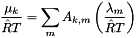where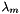is the element potential of element m. The temperature is unchanged. Any phase (ideal or not) that implements this method can be equilibrated by ChemEquil.

Parameters:
 lambda_RT Input vector of dimensionless element potentials The length is equal to nElements().

Reimplemented in ConstDensityThermo, DebyeHuckel, HMWSoln, IdealGasPhase, IdealMolalSoln, IdealSolidSolnPhase, IdealSolnGasVPSS, and MolalityVPSSTP.

Definition at line 1738 of file ThermoPhase.h.

References ThermoPhase::err().

 doublereal size ( int k ) const [inline, inherited]

This routine returns the size of species k.

Parameters:
 k index of the species
Returns:
Returns the size of the species. Units are meters.

Definition at line 309 of file Constituents.h.

References Constituents::m_speciesSize.

 const std::vector< const XML_Node * > & speciesData ( ) const [inherited]

Return a pointer to the vector of XML nodes containing the species data for this phase.

Return a pointer to the XML tree containing the species data for this phase.

Definition at line 950 of file ThermoPhase.cpp.

References Phase::m_kk, and ThermoPhase::m_speciesData.

Referenced by MineralEQ3::initThermoXML(), HMWSoln::initThermoXML(), and DebyeHuckel::initThermoXML().

 bool speciesFrozen ( )  [inline, inherited]

True if freezeSpecies has been called.

Definition at line 318 of file Constituents.h.

References Constituents::m_speciesFrozen.

 int speciesIndex ( std::string name ) const [inherited]

Index of species named 'name'.

The first species added will have index 0, and the last one index nSpecies() - 1.

Parameters:
 name String name of the species
Returns:
Returns the index of the species.

Definition at line 414 of file Constituents.cpp.

References Constituents::m_speciesNames.

 string speciesName ( int k ) const [inherited]
 const vector< string > & speciesNames ( ) const [inherited]

Return a const referernce to the vector of species names.

Definition at line 447 of file Constituents.cpp.

References Constituents::m_speciesNames.

 SpeciesThermo& speciesThermo ( )  [inline, inherited]

Return a changeable reference to the calculation manager for species reference-state thermodynamic properties.

Todo:
This method will fail if no species thermo manager has been installed.

For internal use only.

Reimplemented in DebyeHuckel, HMWSoln, and IdealMolalSoln.

Definition at line 1899 of file ThermoPhase.h.

References ThermoPhase::m_spthermo.

 doublereal standardConcentration ( int k = 0 ) const [virtual]

Return the standard concentration for the kth species.

The standard concentrationused to normalize the activity (i.e., generalized) concentration. This phase assumes that the kinetics operator works on an dimensionless basis. Thus, the standard concentration is equal to 1.0.

Parameters:
 k Optional parameter indicating the species. The default is to assume this refers to species 0.
Returns:
Returns The standard Concentration as 1.0

Reimplemented from ThermoPhase.

Reimplemented in MineralEQ3.

Definition at line 226 of file StoichSubstanceSSTP.cpp.

 int standardStateConvention ( ) const [virtual, inherited]

This method returns the convention used in specification of the standard state, of which there are currently two, temperature based, and variable pressure based.

Currently, there are two standard state conventions:

• Temperature-based activities cSS_CONVENTION_TEMPERATURE 0
• default
• Variable Pressure and Temperature -based activities cSS_CONVENTION_VPSS 1

Reimplemented in VPStandardStateTP.

Definition at line 154 of file ThermoPhase.cpp.

References ThermoPhase::m_ssConvention.

 void stateMFChangeCalc ( bool forceChange = false )  [inline, inherited]

Every time the mole fractions have changed, this routine will increment the stateMFNumber.

Parameters:
 forceChange If this is true then the stateMFNumber always changes. This defaults to false.

Definition at line 31 of file State.cpp.

References State::m_stateNum.

 int stateMFNumber ( ) const [inline, inherited]

Return the state number.

Return the State Mole Fraction Number.

Definition at line 445 of file State.h.

References State::m_stateNum.

 doublereal sum_xlogQ ( doublereal *const Q ) const [inherited]

Evaluate.

Parameters:
 Q Vector of length m_kk to take the log average of
Returns:
Returns the indicated sum.

Definition at line 188 of file State.cpp.

References State::m_mmw, and State::m_ym.

 doublereal sum_xlogx ( ) const [inherited]

Evaluate.

Returns:
returns the indicated sum. units are dimensionless.

Definition at line 184 of file State.cpp.

References State::m_mmw, and State::m_ym.

 doublereal thermalExpansionCoeff ( ) const [virtual]

Return the volumetric thermal expansion coefficient. Units: 1/K.

The thermal expansion coefficient is defined as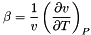Reimplemented from ThermoPhase.

Reimplemented in MineralEQ3.

Definition at line 203 of file StoichSubstanceSSTP.cpp.

 virtual void updateDensity ( )  [inline, virtual, inherited]
Deprecated:

Definition at line 971 of file ThermoPhase.h.

References Cantera::deprecatedMethod().

 virtual doublereal vaporFraction ( ) const [inline, virtual, inherited]

Return the fraction of vapor at the current conditions.

Reimplemented from ThermoPhase.

Definition at line 687 of file SingleSpeciesTP.h.

References SingleSpeciesTP::err().

 XML_Node & xml ( )  [inherited]

Returns a reference to the XML_Node storred for the phase.

The XML_Node for the phase contains all of the input data used to set up the model for the phase, during its initialization.

Definition at line 112 of file Phase.cpp.

References Phase::m_xml.

## Member Data Documentation

 bool m_chargeNeutralityNecessary [protected, inherited]

Boolean indicating whether a charge neutrality condition is a necessity.

Note, the charge neutrality condition is not a necessity for ideal gas phases. There may be a net charge in those phases, because the NASA polynomials for ionized species in Ideal gases take this condition into account. However, liquid phases usually require charge neutrality in order for their derived thermodynamics to be valid.

Definition at line 2130 of file ThermoPhase.h.

 array_fp m_cp0_R [mutable, protected, inherited]

Dimensionless heat capacity at the (mtlast, m_p0).

Definition at line 748 of file SingleSpeciesTP.h.

 Elements* m_Elements [protected, inherited]

Pointer to the element object corresponding to this phase. Normally, this will be the default Element object common to all phases.

Definition at line 366 of file Constituents.h.

 array_fp m_h0_RT [mutable, protected, inherited]

Dimensionless enthalpy at the (mtlast, m_p0).

Definition at line 746 of file SingleSpeciesTP.h.

 bool m_hasElementPotentials [protected, inherited]

Boolean indicating whether there is a valid set of saved element potentials for this phase.

Definition at line 2120 of file ThermoPhase.h.

 int m_index [protected, inherited]

Index number of the phase.

The Cantera interface library uses this member to set the index number to the location of the pointer to this object in the pointer array of ThermoPhase's it maintains. Using this member for any other purpose will lead to unpredictable results if used in conjunction with the interface library.

Reimplemented from Phase.

Definition at line 2106 of file ThermoPhase.h.

Referenced by ThermoPhase::index(), ThermoPhase::operator=(), and ThermoPhase::setIndex().

 vector_fp m_lambdaRRT [protected, inherited]

Vector of element potentials.

-> length equal to number of elements

Definition at line 2116 of file ThermoPhase.h.

 int m_ndim [protected, inherited]

m_ndim is the dimensionality of the phase.

Volumetric phases have dimensionality 3 and surface phases have dimensionality 2.

Definition at line 511 of file Phase.h.

Referenced by Phase::nDim(), Phase::operator=(), and Phase::setNDim().

 doublereal m_p0 [protected, inherited]

Reference pressure (Pa) must be the same for all species

• defaults to 1 atm.

Definition at line 740 of file SingleSpeciesTP.h.

 doublereal m_phi [protected, inherited]

Storred value of the electric potential for this phase.

Units are Volts

Definition at line 2112 of file ThermoPhase.h.

 doublereal m_press [protected, inherited]

The current pressure of the solution (Pa).

It gets initialized to 1 atm.

Definition at line 734 of file SingleSpeciesTP.h.

 array_fp m_s0_R [mutable, protected, inherited]

Dimensionless entropy at the (mtlast, m_p0).

Definition at line 750 of file SingleSpeciesTP.h.

 vector_fp m_speciesComp [protected, inherited]

Atomic composition of the species.

the number of atoms of i in species k is equal to m_speciesComp[k * m_mm + i] The length of this vector is equal to m_kk * m_mm

Definition at line 377 of file Constituents.h.

 std::vector m_speciesData [protected, inherited]

Vector of pointers to the species databases.

This is used to access data needed to construct the transport manager and other properties later in the initialization process. We create a copy of the XML_Node data read in here. Therefore, we own this data.

Definition at line 2096 of file ThermoPhase.h.

 bool m_speciesFrozen [protected, inherited]

Boolean indicating whether the number of species has been frozen.

During the construction of the phase, this is false. After construction of the the phase, this is true.

Definition at line 359 of file Constituents.h.

 std::vector m_speciesNames [protected, inherited]

Vector of the species names.

Definition at line 369 of file Constituents.h.

 vector_fp m_speciesSize [protected, inherited]

m_speciesSize(): Vector of species sizes.

length m_kk This is used in some equations of state which employ the constant partial molar volume approximation. It's so fundamental we've put it at the Constituents class level

Definition at line 393 of file Constituents.h.

 SpeciesThermo* m_spthermo [protected, inherited]

Pointer to the calculation manager for species reference-state thermodynamic properties.

This class is called when the reference-state thermodynamic properties of all the species in the phase needs to be evaluated.

Definition at line 2086 of file ThermoPhase.h.

 int m_ssConvention [protected, inherited]

Contains the standard state convention.

Definition at line 2133 of file ThermoPhase.h.

Referenced by ThermoPhase::operator=(), and ThermoPhase::standardStateConvention().

 doublereal m_tlast [mutable, protected, inherited]

Last temperature used to evaluate the thermodynamic polynomial.

Definition at line 743 of file SingleSpeciesTP.h.

Referenced by SingleSpeciesTP::_updateThermo(), and SingleSpeciesTP::operator=().

 doublereal m_tmax [protected, inherited]

Upper value of the temperature for which reference thermo is valid.

Definition at line 728 of file SingleSpeciesTP.h.

 doublereal m_tmin [protected, inherited]

Lower value of the temperature for which reference thermo is valid.

Definition at line 726 of file SingleSpeciesTP.h.

 vector_fp m_weight [protected, inherited]

Vector of molecular weights of the species.

This vector has length m_kk. The units of the vector are kg kmol-1.

Definition at line 352 of file Constituents.h.

 std::vector xMol_Ref [protected, inherited]

Reference Mole Fraction Composition.

Occasionally, the need arises to find a safe mole fraction vector to initialize the object to. This contains such a vector. The algorithm will pick up the mole fraction vector that is applied from the state xml file in the input file

Definition at line 2142 of file ThermoPhase.h.

The documentation for this class was generated from the following files:
Generated by1.6.3